• ## Python实现多元线性回归

万次阅读 多人点赞 2018-04-12 21:39:25
Python实现多元线性回归 线性回归介绍 线性回归是利用数理统计中回归分析，来确定两种或两种以上变量间相互依赖的定量关系的一种统计分析方法，运用十分广泛。其表达形式为y = w'x+e，e为误差服从均值为0的...
Python实现多元线性回归

线性回归介绍

线性回归是利用数理统计中回归分析，来确定两种或两种以上变量间相互依赖的定量关系的一种统计分析方法，运用十分广泛。其表达形式为y = w'x+e，e为误差服从均值为0的正态分布。回归分析中，只包括一个自变量和一个因变量，且二者的关系可用一条直线近似表示，这种回归分析称为一元线性回归分析。如果回归分析中包括两个或两个以上的自变量，且因变量和自变量之间是线性关系，则称为多元线性回归分析。 线性回归属于回归问题。对于回归问题，解决流程为：  给定数据集中每个样本及其正确答案，选择一个模型函数h（hypothesis，假设），并为h找到适应数据的（未必是全局）最优解，即找出最优解下的h的参数。这里给定的数据集取名叫训练集（Training Set）。不能所有数据都拿来训练，要留一部分验证模型好不好使，这点以后说。先列举几个几个典型的模型： ● 最基本的单变量线性回归：  形如h(x)=theta0+theta1*x1 ● 多变量线性回归：  形如h(x)=theta0+theta1*x1+theta2*x2+theta3*x3 ● 多项式回归（Polynomial Regression）：  形如h(x)=theta0+theta1*x1+theta2*(x2^2)+theta3*(x3^3)  或者h(x)=ttheta0+theta1*x1+theta2*sqr(x2)  但是可以令x2=x2^2，x3=x3^3，于是又将其转化为了线性回归模型。虽然不能说多项式回归问题属于线性回归问题，但是一般我们就是这么做的。 ● 所以最终通用表达式就是：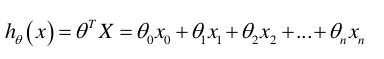数据导入与清洗

在进行数据导入之间需要导入进行线性回归的包：
import pandas as pd
import numpy as np
import matplotlib.pyplot as plt
from pandas import DataFrame,Series
from sklearn.cross_validation import train_test_split
from sklearn.linear_model import LinearRegression
我们利用pandas和numpy对数据进行操作，使用matplotlib进行图像化，使用sklearn进行数据集训练与模型导入。

简单线性回归

对于学生来说，所学习的时间和考试的成绩挂钩，所学习的时间与考试的成绩也是呈线性相关。创建一个数据集来描述学生学习时间与成绩的关系并且做简单的线性回归。

in:
#创建数据集
examDict  = {'学习时间':[0.50,0.75,1.00,1.25,1.50,1.75,1.75,
2.00,2.25,2.50,2.75,3.00,3.25,3.50,4.00,4.25,4.50,4.75,5.00,5.50],
'分数':[10,22,13,43,20,22,33,50,62,
48,55,75,62,73,81,76,64,82,90,93]}

#转换为DataFrame的数据格式
examDf = DataFrame(examDict)

通过DataFrame的函数将字典转化为所需要的数据集，也就是学生成绩与考试成绩的数据集。且关于pandas的数据内容Series与DataFrame可以查看关于pandas的博客了解点击打开链接

out：
  分数  学习时间
0   10  0.50
1   22  0.75
2   13  1.00
3   43  1.25
4   20  1.50
5   22  1.75
6   33  1.75
7   50  2.00
8   62  2.25
9   48  2.50
10  55  2.75
11  75  3.00
12  62  3.25
13  73  3.50
14  81  4.00
15  76  4.25
16  64  4.50
17  82  4.75
18  90  5.00
19  93  5.50

从上面的数据可以看到数据的特征值与其标签，学生所学习的时间就是所需要的特征值，而成绩就是通过特征值所反应的标签。在这个案例中要对数据进行观测来反应学习时间与成绩的情况，将利用散点图来实现简单的观测。

in：
#绘制散点图
plt.scatter(examDf.分数,examDf.学习时间,color = 'b',label = "Exam Data")

#添加图的标签（x轴，y轴）
plt.xlabel("Hours")
plt.ylabel("Score")
#显示图像
plt.show()
out: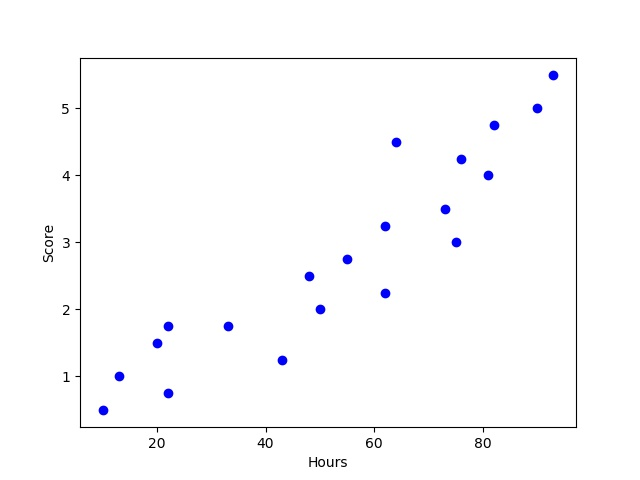从上图可以看到对于分数和时间来说存在相应的线性关系，且俩数据间相关性较强。
在此可以通过相关性来衡量两个变量因素的相关密切程度。
相关系数是用以反映变量之间相关关系密切程度的统计指标。
r(相关系数) = x和y的协方差/(x的标准差*y的标准差) == cov（x,y）/σx*σy（即person系数）
对于相关性强度来说的化有以下的关系：
0~0.3 弱相关
0.3~0.6  中等程度相关
0.6~1  强相关

in：
rDf = examDf.corr()
print(rDf)
out:
分数      学习时间
分数    1.000000  0.923985
学习时间  0.923985  1.000000

pandas中的数学统计函数D.corr()可以反应数据间的相关性关系，可从表值中反应出学习时间与分数之间的相关性为强相关（0.6~1）。对于简单线性回归来来说，简单回归方程为： y = a + b*x (模型建立最佳拟合线)最佳拟合线也是需要通过最小二乘法来实现其作用。对于OLS即最小二乘法我们需要知道的一个关系为点误差，点误差 = 实际值 - 预测值，而误差平方和（Sum of square error） SSE = Σ（实际值-预测值）^2，最小二乘法就是基于SSE实现，最小二乘法 ： 使得误差平方和最小（最佳拟合）。解释完简单线性回归后进行对训练集和测试集的创建，将会使用train_test_split函数来创建（train_test_split是存在与sklearn中的函数）

in：
#将原数据集拆分训练集和测试集
X_train,X_test,Y_train,Y_test = train_test_split(exam_X,exam_Y,train_size=.8)
#X_train为训练数据标签,X_test为测试数据标签,exam_X为样本特征,exam_y为样本标签，train_size 训练数据占比

print("原始数据特征:",exam_X.shape,
",训练数据特征:",X_train.shape,
",测试数据特征:",X_test.shape)

print("原始数据标签:",exam_Y.shape,
",训练数据标签:",Y_train.shape,
",测试数据标签:",Y_test.shape)

#散点图
plt.scatter(X_train, Y_train, color="blue", label="train data")
plt.scatter(X_test, Y_test, color="red", label="test data")

#添加图标标签
plt.legend(loc=2)
plt.xlabel("Hours")
plt.ylabel("Pass")
#显示图像
plt.savefig("tests.jpg")
plt.show()
out:
原始数据特征: (20,) ,训练数据特征: (16,) ,测试数据特征: (4,)
原始数据标签: (20,) ,训练数据标签: (16,) ,测试数据标签: (4,)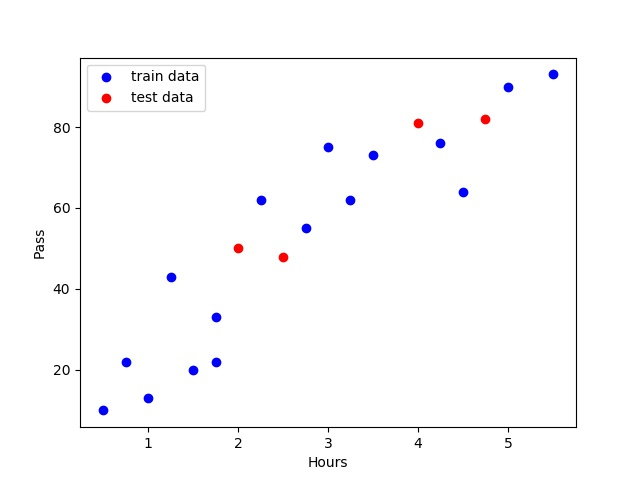tips:由于训练集随机分配的原因每一次运行的结果（点的分布情况，训练集内的情况，测试集内的情况）不都相同在创建数据集之后我们需要将训练集放入skleran中的线性回归模型（LinearRegression()）进行训练，使用函数种的.fit函数进行模型的训练操作。

in：
model = LinearRegression()

#对于模型错误我们需要把我们的训练集进行reshape操作来达到函数所需要的要求
# model.fit(X_train,Y_train)

#reshape如果行数=-1的话可以使我们的数组所改的列数自动按照数组的大小形成新的数组
#因为model需要二维的数组来进行拟合但是这里只有一个特征所以需要reshape来转换为二维数组
X_train = X_train.values.reshape(-1,1)
X_test = X_test.values.reshape(-1,1)

model.fit(X_train,Y_train)

在模型训练完成之后会得到所对应的方程式（线性回归方程式）需要利用函数中的intercept_与coef_来得到

a  = model.intercept_#截距

b = model.coef_#回归系数

print("最佳拟合线:截距",a,",回归系数：",b)

out：

最佳拟合线:截距 7.5580754557 ,回归系数： [ 16.28401865]

由上述的最佳拟合线的截距和回归系数可以算出其线性回归线方程：y = 7.56 + 16.28*x
接下来需要对模型进行预测和对模型进行评价，在进行评价之间将会引入一个决定系数r平方的概念。
对于决定系数R平方常用于评估模型的精确度。
下列为R平方的计算公式：
● y误差平方和 = Σ(y实际值 - y预测值)^2
● y的总波动 = Σ(y实际值 - y平均值)^2
● 有多少百分比的y波动没有被回归拟合线所描述 = SSE/总波动
● 有多少百分比的y波动被回归线描述 = 1 - SSE/总波动 = 决定系数R平方
对于决定系数R平方来说
（1） 回归线拟合程度：有多少百分比的y波动刻印有回归线来描述(x的波动变化)
（2）值大小：R平方越高，回归模型越精确(取值范围0~1)，1无误差，0无法完成拟合对于预测来说我们需要运用函数中的model.predict()来得到预测值

in：
#训练数据的预测值
y_train_pred = model.predict(X_train)
#绘制最佳拟合线：标签用的是训练数据的预测值y_train_pred
plt.plot(X_train, y_train_pred, color='black', linewidth=3, label="best line")

#测试数据散点图
plt.scatter(X_test, Y_test, color='red', label="test data")

#添加图标标签
plt.legend(loc=2)
plt.xlabel("Hours")
plt.ylabel("Score")
#显示图像
plt.savefig("lines.jpg")
plt.show()

score = model.score(X_test,Y_test)

print(score)

out:
score : 0.834706696876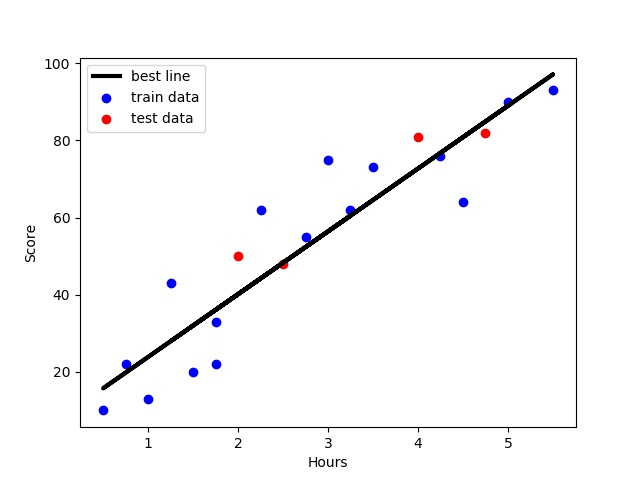多元线性回归

in：
#通过read_csv来读取我们的目的数据集
#清洗不需要的数据
#得到我们所需要的数据集且查看其前几列以及数据形状
print('head:',new_adv_data.head(),'\nShape:',new_adv_data.shape)
out:
head:       TV  radio  newspaper  sales
0  230.1   37.8       69.2   22.1
1   44.5   39.3       45.1   10.4
2   17.2   45.9       69.3    9.3
3  151.5   41.3       58.5   18.5
4  180.8   10.8       58.4   12.9
Shape:  (200, 4)

对于上述的数据可以得到数据中
标签值（sales）：
Sales：对应产品的销量
在这个案例中，通过不同的广告投入，预测产品销量。因为响应变量是一个连续的值，所以这个问题是一个回归问题。数据集一共有200个观测值，每一组观测对应一个市场的情况。接下里对数据进行描述性统计，以及寻找缺失值（缺失值对模型的影响较大，如发现缺失值应替换或删除）,且利用箱图来从可视化方面来查看数据集，在描述统计之后对数据进行相关性分析，以此来查找数据中特征值与标签值之间的关系。

in：
#数据描述
#缺失值检验

plt.savefig("boxplot.jpg")
plt.show()
##相关系数矩阵 r(相关系数) = x和y的协方差/(x的标准差*y的标准差) == cov（x,y）/σx*σy
#相关系数0~0.3弱相关0.3~0.6中等程度相关0.6~1强相关
print(new_adv_data.corr())

out:
               TV       radio   newspaper       sales
count  200.000000  200.000000  200.000000  200.000000
mean   147.042500   23.264000   30.554000   14.022500
std     85.854236   14.846809   21.778621    5.217457
min      0.700000    0.000000    0.300000    1.600000
25%     74.375000    9.975000   12.750000   10.375000
50%    149.750000   22.900000   25.750000   12.900000
75%    218.825000   36.525000   45.100000   17.400000
max    296.400000   49.600000  114.000000   27.000000
TV           0
newspaper    0
sales        0
dtype: int64
TV         1.000000  0.054809   0.056648  0.782224
newspaper  0.056648  0.354104   1.000000  0.228299
sales      0.782224  0.576223   0.228299  1.000000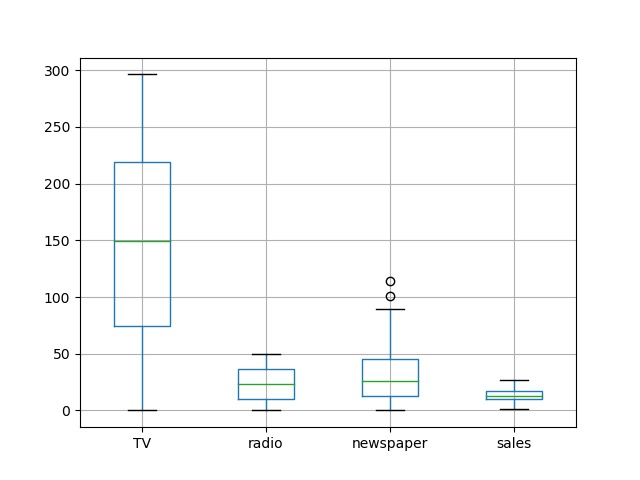in：
# 通过加入一个参数kind='reg'，seaborn可以添加一条最佳拟合直线和95%的置信带。
plt.savefig("pairplot.jpg")
plt.show()
out: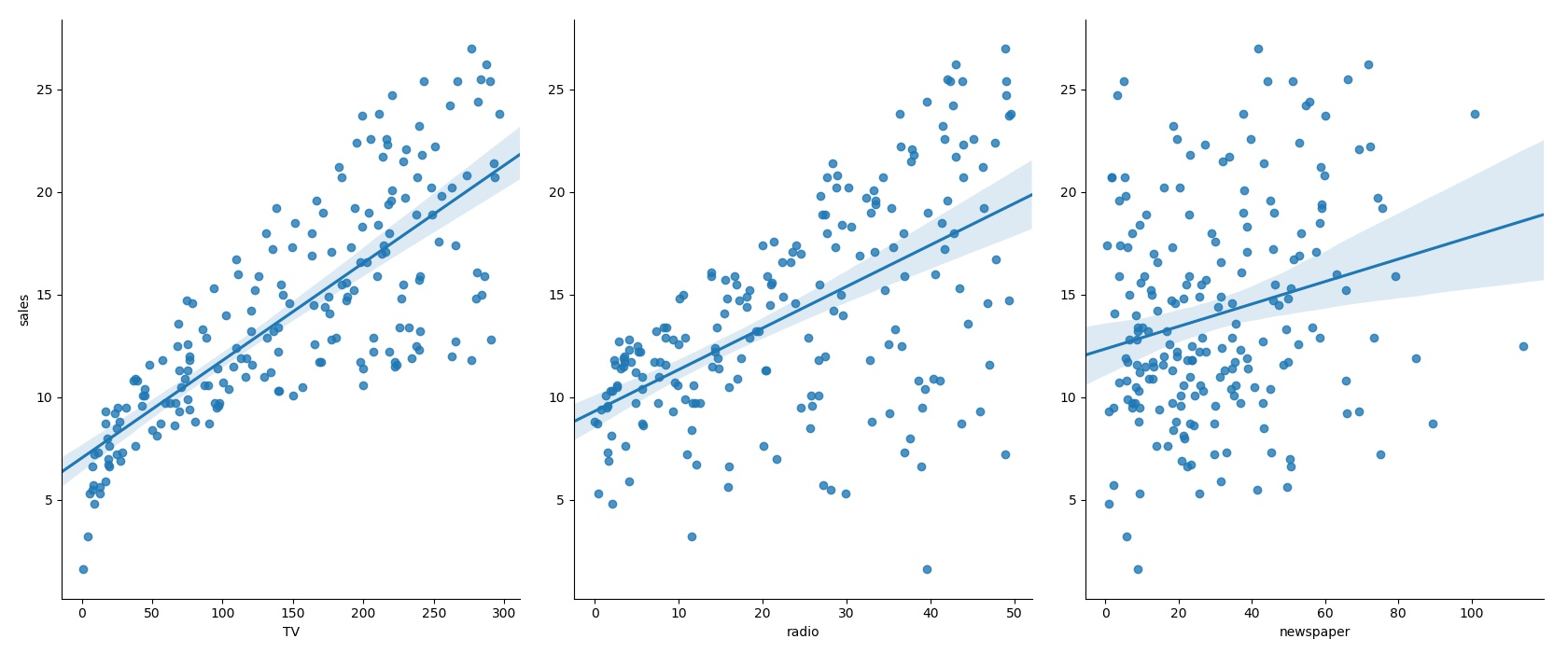上如图种所示，可以了解到不同的因素对销量的预测线（置信度= 95 %），也可可以大致看出不同特征对于标签值的影响与相关关系在了解了数据的各种情况后需要对数据集建立模型，在建立模型的第一步我们将建立训练集与测试集同样的将会使用train_test_split函数来创建（train_test_split是存在与sklearn中的函数）

in：
X_train,X_test,Y_train,Y_test = train_test_split(new_adv_data.ix[:,:3],new_adv_data.sales,train_size=.80)

",训练数据特征:",X_train.shape,
",测试数据特征:",X_test.shape)

",训练数据标签:",Y_train.shape,
",测试数据标签:",Y_test.shape)
out:
原始数据特征: (200, 3) ,训练数据特征: (160, 3) ,测试数据特征: (40, 3)
原始数据标签: (200,) ,训练数据标签: (160,) ,测试数据标签: (40,)

建立初步的数据集模型之后将训练集中的特征值与标签值放入LinearRegression()模型中且使用fit函数进行训练,在模型训练完成之后会得到所对应的方程式（线性回归方程式）需要利用函数中的intercept_与coef_。

in：
model = LinearRegression()

model.fit(X_train,Y_train)

a  = model.intercept_#截距

b = model.coef_#回归系数

print("最佳拟合线:截距",a,",回归系数：",b)

out:
最佳拟合线:截距 2.79361553401 ,回归系数： [ 0.04711495  0.18719875 -0.00185999]

即所得的多元线性回归模型的函数为 ： y = 2.79 + 0.04 * TV + 0.187 * Radio - 0.002 * Newspaper,对于给定了Radio和Newspaper的广告投入，如果在TV广告上每多投入1个单位，对应销量将增加0.04711个单位。就是加入其它两个媒体投入固定，在TV广告上每增加1000美元（因为单位是1000美元），销量将增加47.11（因为单位是1000）。但是大家注意这里的newspaper的系数居然是负数，所以我们可以考虑不使用newspaper这个特征。接下来对数据集进行预测与模型测评。同样使用predict与score函数来获取所需要的预测值与得分。

in：
#R方检测
#决定系数r平方
#对于评估模型的精确度
#y误差平方和 = Σ(y实际值 - y预测值)^2
#y的总波动 = Σ(y实际值 - y平均值)^2
#有多少百分比的y波动没有被回归拟合线所描述 = SSE/总波动
#有多少百分比的y波动被回归线描述 = 1 - SSE/总波动 = 决定系数R平方
#对于决定系数R平方来说1） 回归线拟合程度：有多少百分比的y波动刻印有回归线来描述(x的波动变化)
#2）值大小：R平方越高，回归模型越精确(取值范围0~1)，1无误差，0无法完成拟合
score = model.score(X_test,Y_test)

print(score)

#对线性回归进行预测

Y_pred = model.predict(X_test)

print(Y_pred)

plt.plot(range(len(Y_pred)),Y_pred,'b',label="predict")
#显示图像
plt.savefig("predict.jpg")
plt.show()

out:
score : 0.871755480886
predict :[ 14.17217173  17.42866884  16.81933374  18.16079802   7.64784604
17.8670496   16.66488531  14.98782916   9.41023763  16.21679696
19.32696651   7.76788593  23.34231219   3.59006148  13.15777984
24.26609169  15.47571902  15.39542342  13.98430709  12.65446708
7.59818691  13.85179898  12.16325619  10.34902817  11.9813427
11.05726513   8.13405159  21.94038306  16.4388483   14.06506403
4.36052153   6.45326681   7.55083036  24.25987365  17.13603444
14.04814117   7.28664465  17.24163581  20.42745536   6.55512244]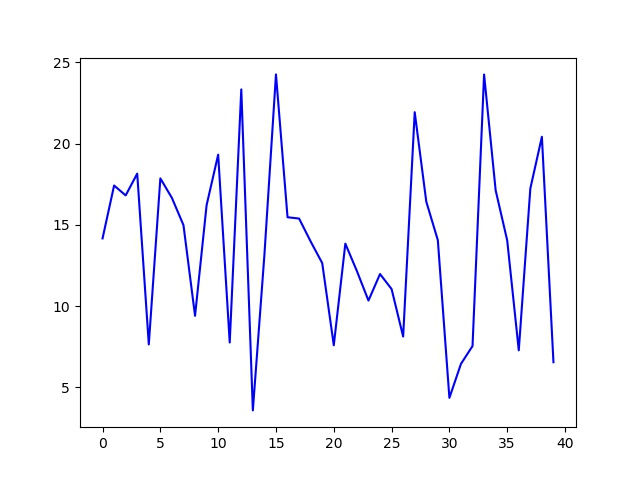预测集与源数据集的对比如下：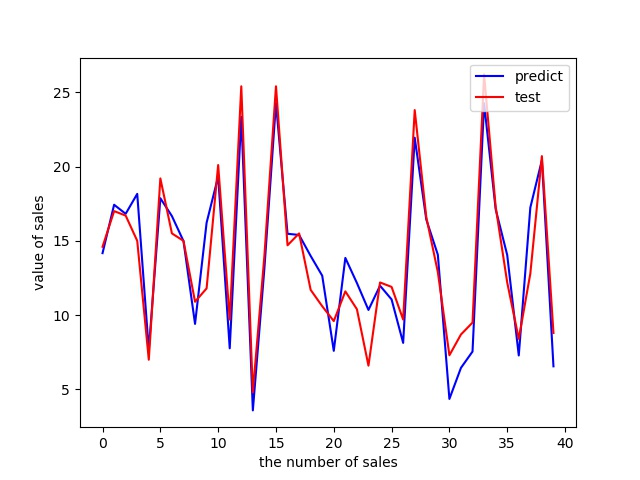模型的检测方法-ROC曲线：
ROC曲线是根据一系列不同的二分类方式（分界值或决定阈），以真阳性率（灵敏度）为纵坐标，假阳性率（1-特异度）为横坐标绘制的曲线。传统的诊断试验评价方法有一个共同的特点，必须将试验结果分为两类，再进行统计分析。ROC曲线的评价方法与传统的评价方法不同，无须此限制，而是根据实际情况，允许有中间状态，可以把试验结果划分为多个有序分类，如正常、大致正常、可疑、大致异常和异常五个等级再进行统计分析。因此，ROC曲线评价方法适用的范围更为广泛。
1.ROC曲线能很容易地查出任意界限值时的对疾病的识别能力。
2.选择最佳的诊断界限值。ROC曲线越靠近左上角,试验的准确性就越高。最靠近左上角的ROC曲线的点是错误最少的最好阈值，其假阳性和假阴性的总数最少。
3.两种或两种以上不同诊断试验对疾病识别能力的比较。在对同一种疾病的两种或两种以上诊断方法进行比较时，可将各试验的ROC曲线绘制到同一坐标中，以直观地鉴别优劣，靠近左上角的ROC曲线所代表的受试者工作最准确。亦可通过分别计算各个试验的ROC曲线下的面积(AUC)进行比较，哪一种试验的 AUC最大，则哪一种试验的诊断价值最佳(百度百科)
模型提升：
对于提升模型准确度的方法很多，在这个模型下，可以利用异常值替换，将Newspaper中的异常值进行拉格朗日法插补，朗格朗日插补法（from scipy.interpolate import lagrange即scipy中的函数）可以间接提高模型的准确度，如果不需要插补异常值或缺失值的话可以将Newspaper不列为特征值考虑，在不考虑Newspaper为特征值的情况下，新的模型的准确率将超过旧模型，也可以从模型的准确度来反证Newspaper不适合作为特征值。

整体代码如下，数据集再上面链接中可直接下载。
import pandas as pd
import numpy as np
import matplotlib.pyplot as plt
from pandas import DataFrame,Series
from sklearn.cross_validation import train_test_split
from sklearn.linear_model import LinearRegression
#创建数据集
examDict  = {'学习时间':[0.50,0.75,1.00,1.25,1.50,1.75,1.75,
2.00,2.25,2.50,2.75,3.00,3.25,3.50,4.00,4.25,4.50,4.75,5.00,5.50],
'分数':[10,22,13,43,20,22,33,50,62,
48,55,75,62,73,81,76,64,82,90,93]}

#转换为DataFrame的数据格式
examDf = DataFrame(examDict)

#绘制散点图
plt.scatter(examDf.分数,examDf.学习时间,color = 'b',label = "Exam Data")

#添加图的标签（x轴，y轴）
plt.xlabel("Hours")
plt.ylabel("Score")
#显示图像
plt.savefig("examDf.jpg")
plt.show()

#相关系数矩阵 r(相关系数) = x和y的协方差/(x的标准差*y的标准差) == cov（x,y）/σx*σy
#相关系数0~0.3弱相关0.3~0.6中等程度相关0.6~1强相关
rDf = examDf.corr()
print(rDf)

#回归方程 y = a + b*x (模型建立最佳拟合线)
#点误差 = 实际值 - 拟合值
#误差平方和（Sum of square error） SSE = Σ（实际值-预测值）^2
#最小二乘法 ： 使得误差平方和最小（最佳拟合）
exam_X  =  examDf.loc[:,'学习时间']
exam_Y  =  examDf.loc[:,'分数']

#将原数据集拆分训练集和测试集
X_train,X_test,Y_train,Y_test = train_test_split(exam_X,exam_Y,train_size=.8)
#X_train为训练数据标签,X_test为测试数据标签,exam_X为样本特征,exam_y为样本标签，train_size 训练数据占比

print("原始数据特征:",exam_X.shape,
",训练数据特征:",X_train.shape,
",测试数据特征:",X_test.shape)

print("原始数据标签:",exam_Y.shape,
",训练数据标签:",Y_train.shape,
",测试数据标签:",Y_test.shape)

#散点图
plt.scatter(X_train, Y_train, color="blue", label="train data")
plt.scatter(X_test, Y_test, color="red", label="test data")

#添加图标标签
plt.legend(loc=2)
plt.xlabel("Hours")
plt.ylabel("Pass")
#显示图像
plt.savefig("tests.jpg")
plt.show()

model = LinearRegression()

#对于下面的模型错误我们需要把我们的训练集进行reshape操作来达到函数所需要的要求
# model.fit(X_train,Y_train)

#reshape如果行数=-1的话可以使我们的数组所改的列数自动按照数组的大小形成新的数组
#因为model需要二维的数组来进行拟合但是这里只有一个特征所以需要reshape来转换为二维数组
X_train = X_train.values.reshape(-1,1)
X_test = X_test.values.reshape(-1,1)

model.fit(X_train,Y_train)

a  = model.intercept_#截距

b = model.coef_#回归系数

print("最佳拟合线:截距",a,",回归系数：",b)

#决定系数r平方
#对于评估模型的精确度
#y误差平方和 = Σ(y实际值 - y预测值)^2
#y的总波动 = Σ(y实际值 - y平均值)^2
#有多少百分比的y波动没有被回归拟合线所描述 = SSE/总波动
#有多少百分比的y波动被回归线描述 = 1 - SSE/总波动 = 决定系数R平方
#对于决定系数R平方来说1） 回归线拟合程度：有多少百分比的y波动刻印有回归线来描述(x的波动变化)
#2）值大小：R平方越高，回归模型越精确(取值范围0~1)，1无误差，0无法完成拟合

plt.scatter(X_train, Y_train, color='blue', label="train data")

#训练数据的预测值
y_train_pred = model.predict(X_train)
#绘制最佳拟合线：标签用的是训练数据的预测值y_train_pred
plt.plot(X_train, y_train_pred, color='black', linewidth=3, label="best line")

#测试数据散点图
plt.scatter(X_test, Y_test, color='red', label="test data")

#添加图标标签
plt.legend(loc=2)
plt.xlabel("Hours")
plt.ylabel("Score")
#显示图像
plt.savefig("lines.jpg")
plt.show()

score = model.score(X_test,Y_test)

print(score)
import pandas as pd
import seaborn as sns
from sklearn.linear_model import LinearRegression
import matplotlib.pyplot as plt
from sklearn.cross_validation import train_test_split

#清洗不需要的数据
#得到我们所需要的数据集且查看其前几列以及数据形状

#数据描述
#缺失值检验

plt.savefig("boxplot.jpg")
plt.show()
##相关系数矩阵 r(相关系数) = x和y的协方差/(x的标准差*y的标准差) == cov（x,y）/σx*σy
#相关系数0~0.3弱相关0.3~0.6中等程度相关0.6~1强相关

#建立散点图来查看数据集里的数据分布
#seaborn的pairplot函数绘制X的每一维度和对应Y的散点图。通过设置size和aspect参数来调节显示的大小和比例。
# 通过加入一个参数kind='reg'，seaborn可以添加一条最佳拟合直线和95%的置信带。
plt.savefig("pairplot.jpg")
plt.show()

#利用sklearn里面的包来对数据集进行划分，以此来创建训练集和测试集
#train_size表示训练集所占总数据集的比例

",训练数据特征:",X_train.shape,
",测试数据特征:",X_test.shape)

",训练数据标签:",Y_train.shape,
",测试数据标签:",Y_test.shape)

model = LinearRegression()

model.fit(X_train,Y_train)

a  = model.intercept_#截距

b = model.coef_#回归系数

print("最佳拟合线:截距",a,",回归系数：",b)

#R方检测
#决定系数r平方
#对于评估模型的精确度
#y误差平方和 = Σ(y实际值 - y预测值)^2
#y的总波动 = Σ(y实际值 - y平均值)^2
#有多少百分比的y波动没有被回归拟合线所描述 = SSE/总波动
#有多少百分比的y波动被回归线描述 = 1 - SSE/总波动 = 决定系数R平方
#对于决定系数R平方来说1） 回归线拟合程度：有多少百分比的y波动刻印有回归线来描述(x的波动变化)
#2）值大小：R平方越高，回归模型越精确(取值范围0~1)，1无误差，0无法完成拟合
score = model.score(X_test,Y_test)

print(score)

#对线性回归进行预测

Y_pred = model.predict(X_test)

print(Y_pred)

plt.plot(range(len(Y_pred)),Y_pred,'b',label="predict")
#显示图像
# plt.savefig("predict.jpg")
plt.show()

plt.figure()
plt.plot(range(len(Y_pred)),Y_pred,'b',label="predict")
plt.plot(range(len(Y_pred)),Y_test,'r',label="test")
plt.legend(loc="upper right") #显示图中的标签
plt.xlabel("the number of sales")
plt.ylabel('value of sales')
plt.savefig("ROC.jpg")
plt.show()


TVradionewspapersales230.137.869.222.144.539.345.110.417.245.969.39.3151.541.358.518.5180.810.858.412.98.748.9757.257.532.823.511.8120.219.611.613.28.62.114.8199.82.621.210.666.15.824.28.6214.724417.423.835.165.99.297.57.67.29.7204.132.94619195.447.752.922.467.836.611412.5281.439.655.824.469.220.518.311.3147.323.919.114.6218.427.753.418237.45.123.512.513.215.949.65.6228.316.926.215.562.312.618.39.7262.93.519.512142.929.312.615240.116.722.915.9248.827.122.918.970.61640.810.5292.928.343.221.4112.917.438.611.997.21.5309.6265.6200.317.495.71.47.49.5290.74.18.512.8266.943.8525.474.749.445.714.743.126.735.110.122837.73221.5202.522.331.616.617733.438.717.1293.627.71.820.7206.98.426.412.925.125.743.38.5175.122.531.514.989.79.935.710.6239.941.518.523.2227.215.849.914.866.911.736.89.7199.83.134.611.4100.49.63.610.7216.441.739.622.6182.646.258.721.2262.728.815.920.2198.949.46023.77.328.141.45.5136.219.216.613.2210.849.637.723.8210.729.59.318.453.5221.48.1261.342.754.724.2239.315.527.315.7102.729.68.414131.142.828.918699.30.99.331.524.62.29.5139.314.510.213.4237.427.51118.9216.843.927.222.3199.130.638.718.3109.814.331.712.426.83319.38.8129.45.731.311213.424.613.11716.943.789.48.727.51.620.76.9120.528.514.214.25.429.99.45.31167.723.11176.426.722.311.8239.84.136.912.375.320.332.511.368.444.535.613.6213.54333.821.7193.218.465.715.276.327.51612110.740.663.21688.325.573.412.9109.847.851.416.7134.34.99.311.228.61.5337.3217.733.55919.4250.936.572.322.2107.41410.911.5163.331.652.916.9197.63.55.911.7184.9212215.5289.742.351.225.4135.241.745.917.2222.44.349.811.7296.436.3100.923.8280.210.121.414.8187.917.217.914.7238.234.35.320.7137.946.45919.2251129.77.290.40.323.28.713.10.425.65.3255.426.95.519.8225.88.256.513.4241.73823.221.8175.715.42.414.1209.620.610.715.978.246.834.514.675.13552.712.6139.214.325.612.276.40.814.89.4125.736.979.215.919.41622.36.6141.326.846.215.518.821.750.472242.415.611.6123.134.612.415.2229.532.374.219.787.211.825.910.67.838.950.66.680.209.28.8220.3493.224.759.61243.19.70.739.68.71.6265.22.94312.78.427.22.15.7219.833.545.119.636.938.665.610.848.3478.511.625.6399.39.5273.728.959.720.84325.920.59.6184.943.91.720.773.41712.910.9193.735.475.619.2220.533.237.920.1104.65.734.410.496.214.838.911.4140.31.9910.3240.17.38.713.2243.24944.325.43840.311.910.944.725.820.610.1280.713.93716.11218.448.711.6197.623.314.216.6171.339.737.719187.821.19.515.64.111.65.73.293.943.550.515.3149.81.324.310.111.736.945.27.3131.718.434.612.9172.518.130.714.485.735.849.313.3188.418.125.614.9163.536.87.418117.214.75.411.9234.53.484.811.917.937.621.68206.85.219.412.2215.423.657.617.1284.310.66.4155011.618.48.4164.520.947.414.519.620.1177.6168.47.112.811.7222.43.413.111.5276.948.941.827248.430.220.320.2170.27.835.211.7276.72.323.711.8165.61017.612.6156.62.68.310.5218.55.427.412.256.25.729.78.7287.64371.826.2253.821.33017.620545.119.622.6139.52.126.610.3191.128.718.217.328613.93.715.918.712.123.46.739.541.15.810.875.510.869.917.24.131.65.9166.8423.619.6149.735.6617.338.23.713.87.694.24.98.19.71779.36.412.8283.64266.225.5232.18.68.713.4
展开全文Python 数据分析 数据挖掘 pandas
• 1. SPSS当存在分类变量时，需要首先处理成多个哑变量，例如将年龄分成三个:age60。然后在SPSS里纳入除了ref的所有哑变量，例如我们要将18-60作为参考组，就纳入age60，不要纳入age18-60。首先可以选择stepwise进行...
1. SPSS
当存在分类变量时，需要首先处理成多个哑变量，例如将年龄分成三个:age<18, age18_60, age>60。
然后在SPSS里纳入除了ref的所有哑变量，例如我们要将18-60作为参考组，就纳入age<18和age>60，不要纳入age18-60。
首先可以选择stepwise进行特征筛选建模。但是勾选逐步后，不能enable Bootstrap。所以可以分两步走，第一步逐步来筛选变量。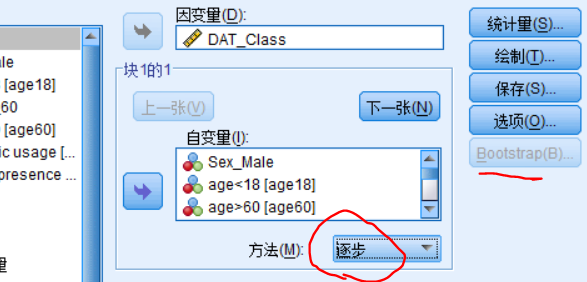然后根据要纳入的变量，再执行一次回归，选择进入并且勾选bootstrap。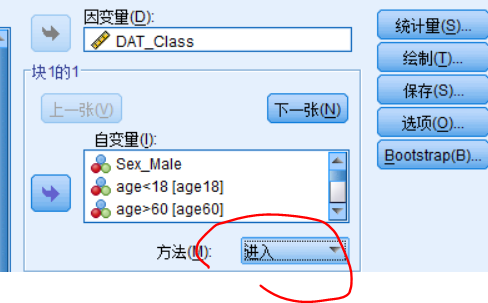2. Python
python中的statsmodels可以
from statsmodels.formula.api import ols
formula = f"DAT_Class~C(Sex)+C(年龄_分类)+C(Antibiotic_usage)+C(Tumor_presence)+C(Trans)+贫血+消化+低血容量+感染+凝血障碍+肺炎+脓毒血症+休克+急性白血病+糖尿病+早产儿+const"
model = ols(formula, data=df).fit()
model.summary()
利用这个方式也可以对分类变量指定参考组。例如指定年龄的参考组为18-60：
formula = f"DAT_Class~C(Sex)+C(年龄_分类, Treatment(reference='18-60'))+C(Antibiotic_usage)+C(Tumor_presence)+C(Trans)+贫血+消化+低血容量+感染+凝血障碍+肺炎+脓毒血症+休克+急性白血病+糖尿病+早产儿+const"
展开全文• 多元线性回归是研究一个连续型变量和其他多个变量间线性关系的统计学分析方法，如果在自变量中存在分类变量，如果直接将分类变量和连续性变量统一纳入模型进行分析是有问题的，尤其是无序分类资料，即使进入了模型，...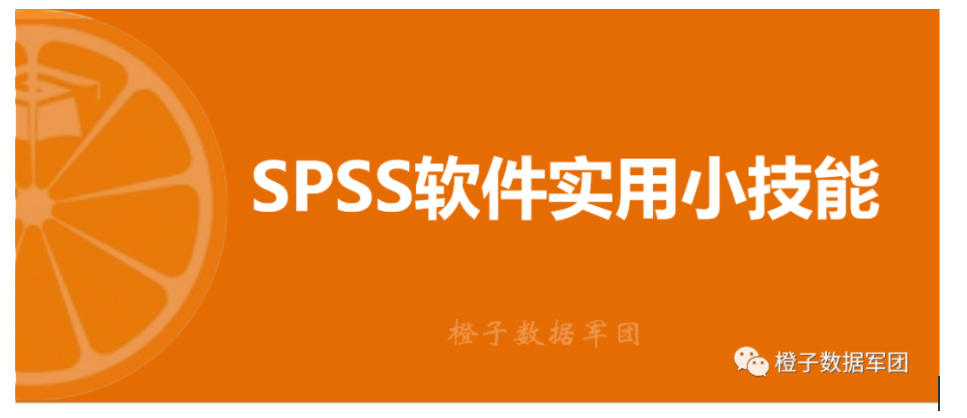多元线性回归是研究一个连续型变量和其他多个变量间线性关系的统计学分析方法，如果在自变量中存在分类变量，如果直接将分类变量和连续性变量统一纳入模型进行分析是有问题的，尤其是无序分类资料，即使进入了模型，也难以解释，因此分类资料纳入模型最佳的方式是设置哑变量。
在SPSS软件中，做Logistic回归时，直接有选项可以将分类变量设置哑变量，但是在做多元线性回归时，分析过程中没有设置哑变量的选项，就需要对原始数据进行拆解，将分类变量拆解成哑变量的形式。（值得一提的是，如果应变量能够转化为二分类的变量，直接采用Logistic回归分析也可以直接分析）
下面介绍在SPSS软件中多元线性回归哑变量设置的方法
以模拟的脑卒中患者康复期生活质量影响因素分析的数据为例，脑卒中患者的生活质量采用卒中专门生存质量量表（SS-QOL）来体现，SS-QOL评分是一个连续性资料，其分数越高，生存质量越好，为探究其影响因素，纳入以下一些研究变量：年龄、婚姻状况、文化程度、职业、BI评分。下图是数据格式，可以看出婚姻状况、文化程度、职业是分类资料。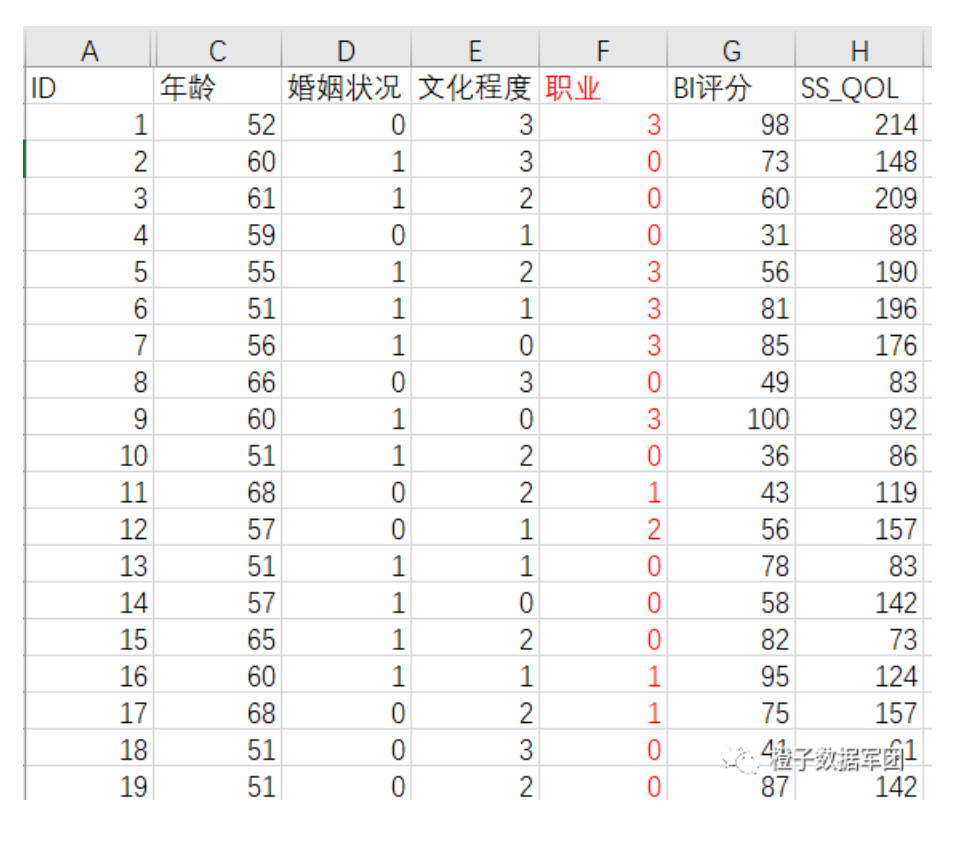从变量的赋值来看，这三个分类资料是无序分类资料，分析时，需要设置哑变量。下面介绍哑变量设置的方法。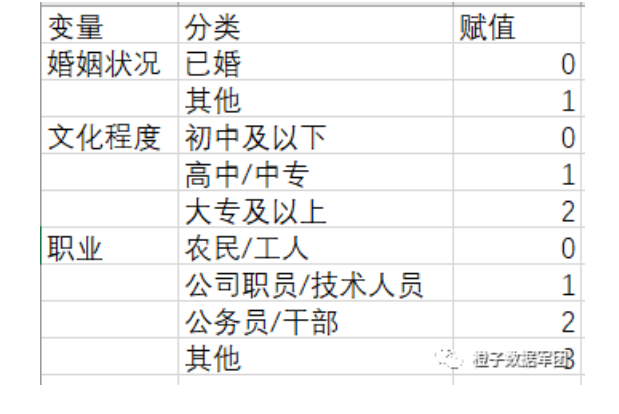第一步 SPSS菜单栏中 转换-创建虚变量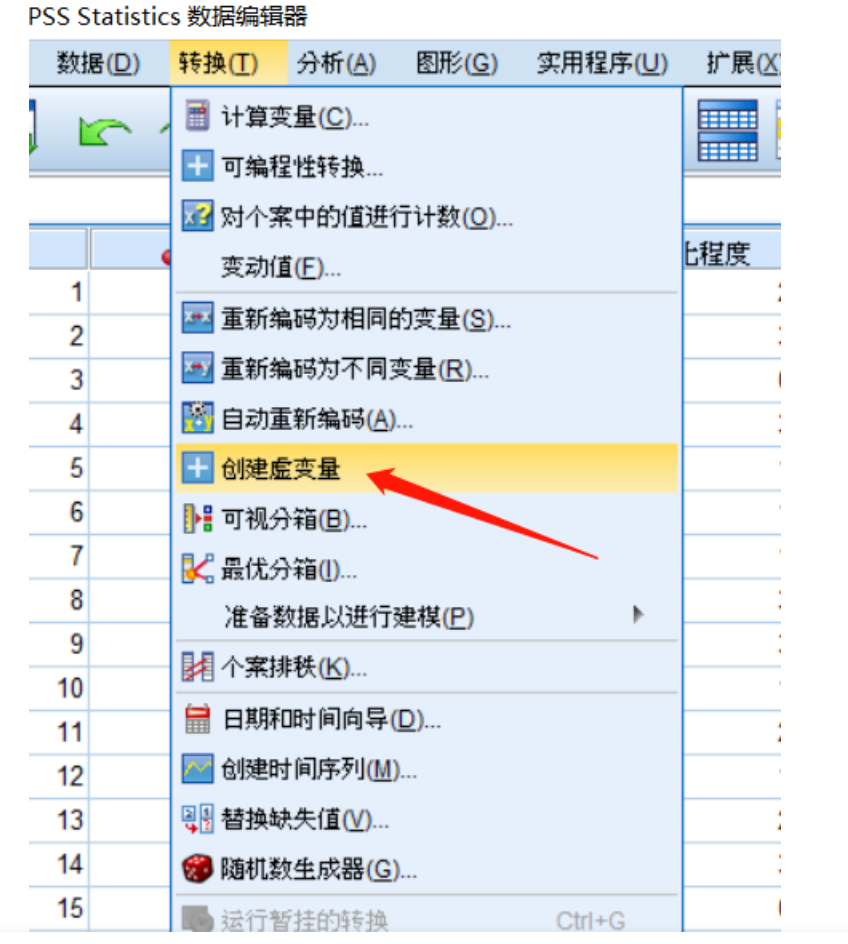第二步 选择分类变量，将其放入“针对下列变量创建虚变量”框里，再重新命名哑变量的名称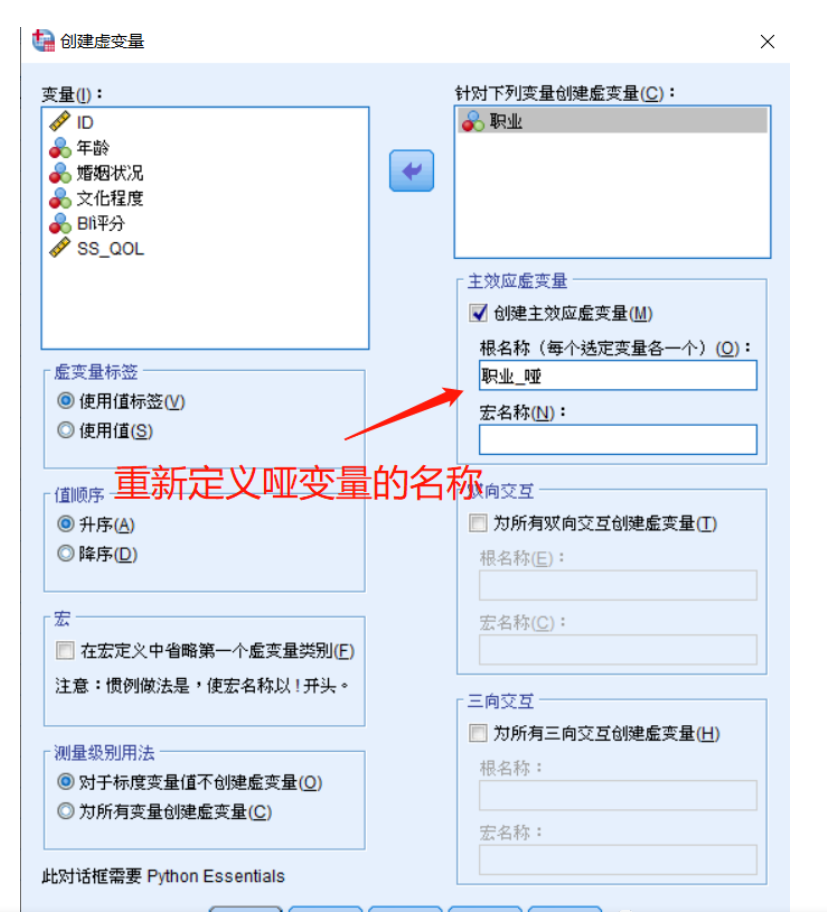即可在数据中看到创建的哑变量，职业有4种分类，因此创建了4个哑变量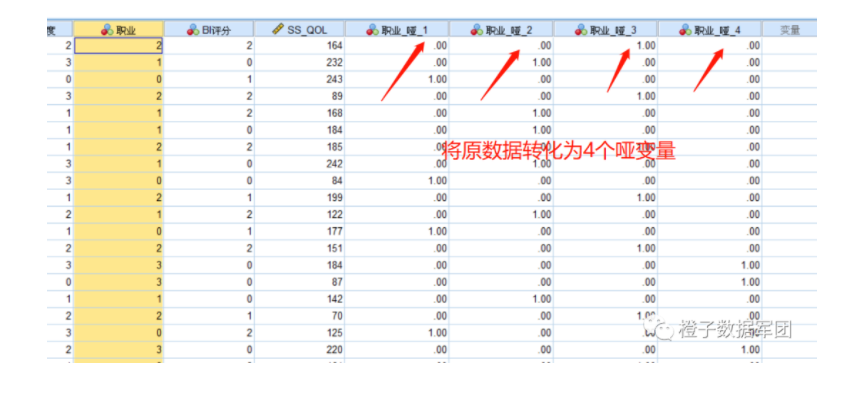按照同样的方法，将其他分类变量创建哑变量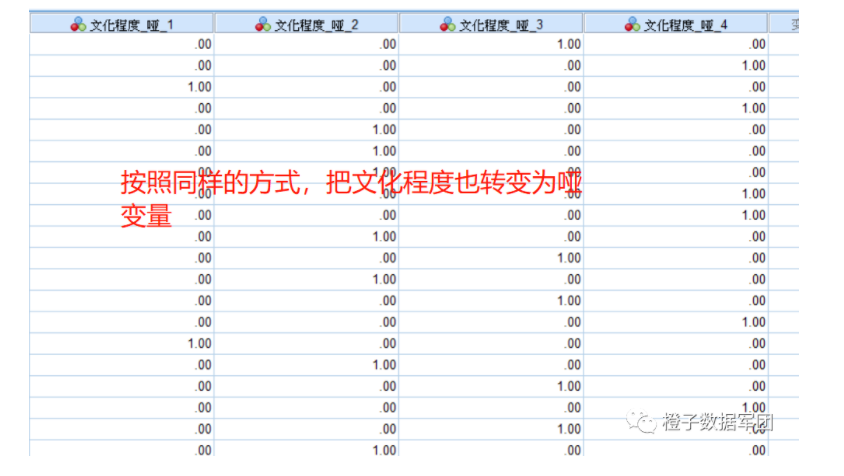第三步 进行多元线性回归分析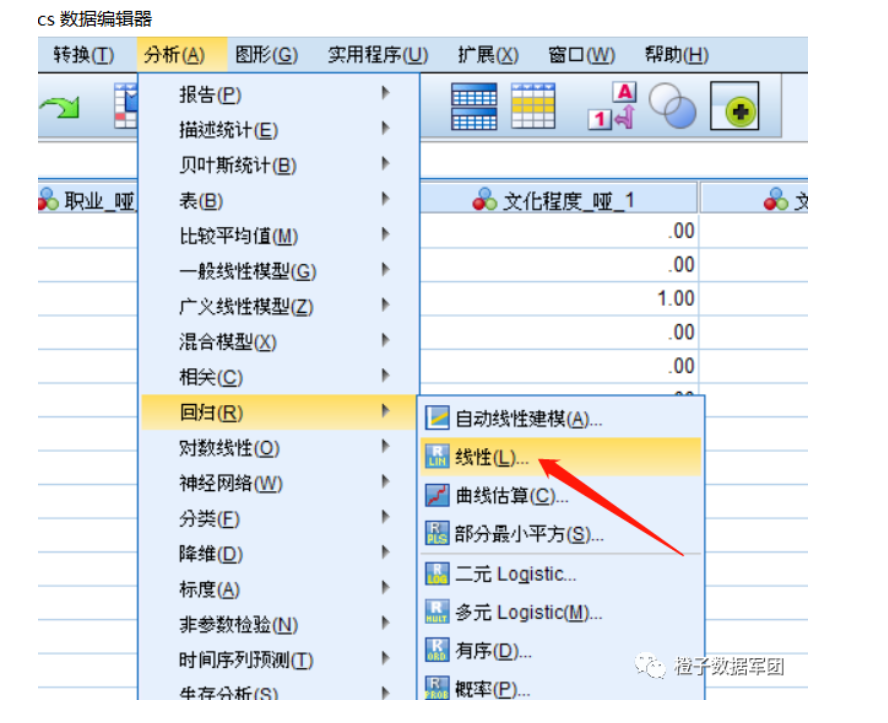第四步 哑变量选择
这里注意的是，分类变量的哑变量中确定一个参照变量，然后将除参考变量以外的其他哑变量同时放入自变量框中，如下图所示，把职业=0的做为参照，其他3个哑变量放入模型。
此外，由于哑变量要同出同进模型，因此方法必须选择“输入”
设置好一个哑变量后点“下一个”设置另一个哑变量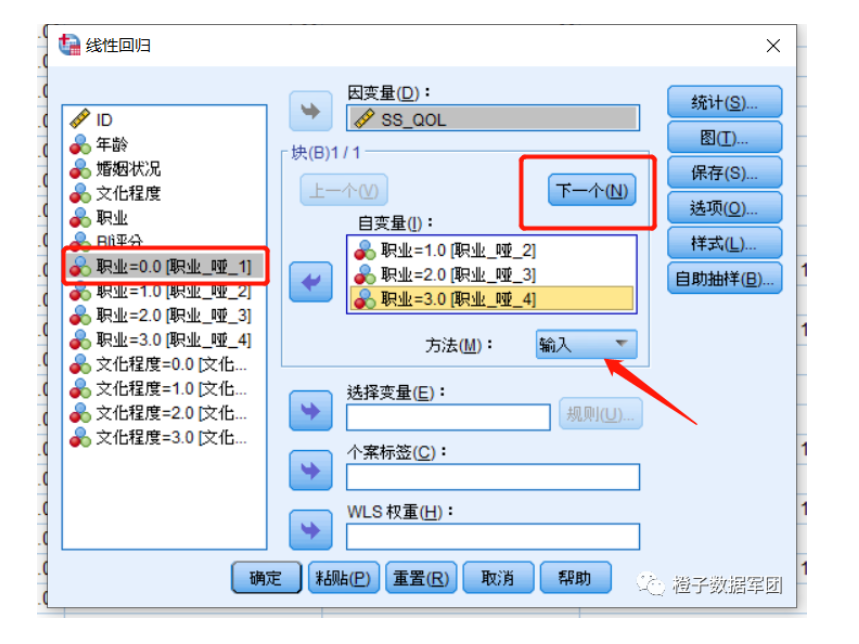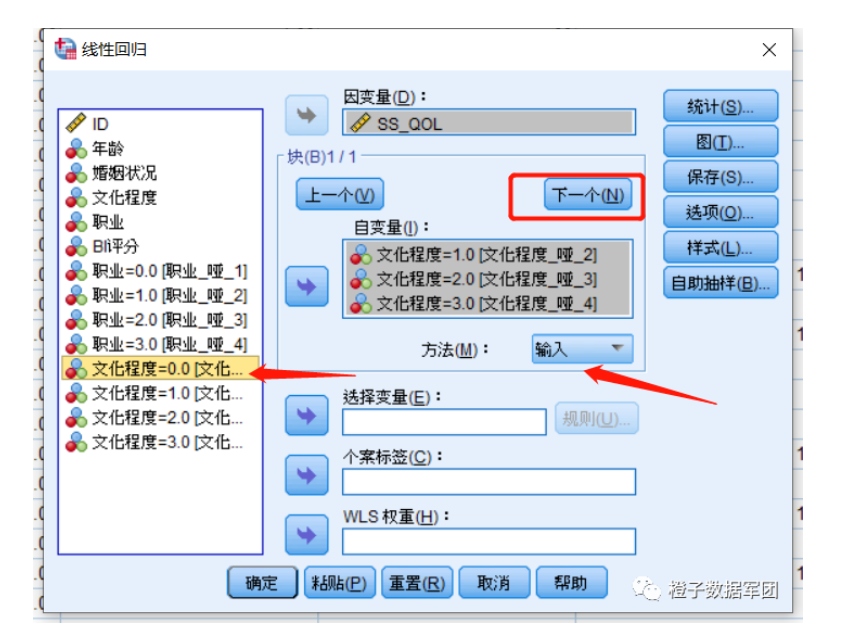以此类推，先把所有分类变量设置好
第五步 放置其余变量
这个时候，其他的变量进入模型的方法就可以自由选择了，可以选择逐步，也可以选择其他。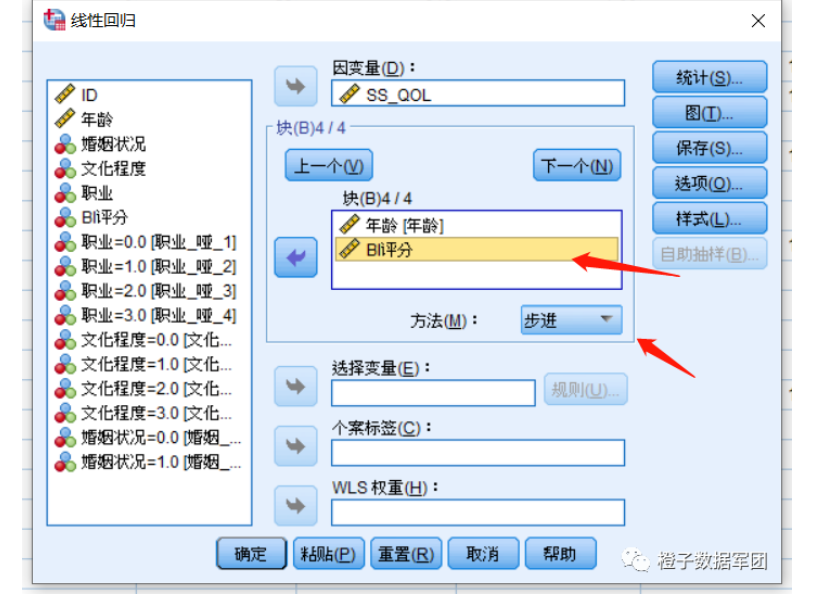以上就是多元线性回归哑变量的设置方法。即使解决了哑变量的设置问题，在多元线性回归中，由于哑变量要同进同出，如果有多个分类变量的话，这些多分类是用软件没有办法来进行逐步回归，只能手动选择分类变量进入模型，多次比较模型效果来确定，此外，哑变量的参照组选择不同，对模型结果也是有影响，因此在设置参照哑变量时，可以进行多次尝试，选择对模型解释最佳的参照哑变量。 获取详细教学视频及粉丝交流群，请关注微信公众号【橙子数据军团】展开全文• 多元线性回归 pythonLinear regression is a standard statistical data analysis technique. We use linear regression to determine the direct relationship between a dependent variable and one or more ...

多元线性回归 python

Linear regression is a standard statistical data analysis technique. We use linear regression to determine the direct relationship between a dependent variable and one or more independent variables. The dependent variable must be measured on a continuous measurement scale, and the independent variable(s) can be measured on either a categorical or continuous measurement scale.
线性回归是一种标准的统计数据分析技术。 我们使用线性回归来确定因变量和一个或多个自变量之间的直接关系。 因变量必须在连续测量范围内进行测量，并且自变量可以在分类或连续测量范围内进行测量。
In linear regression, we want to draw a line that comes closest to the data by finding the slope and intercept, which define the line and minimize regression errors. There are two types of linear regression: simple linear regression and multiple linear regression. In this note, we will focus on multiple linear regression.
在线性回归中，我们希望通过找到斜率和截距来画一条最接近数据的线，以定义直线并使回归误差最小。 线性回归有两种类型：简单线性回归和多重线性回归。 在本文中，我们将重点介绍多元线性回归。
The structure of this note:
本注释的结构：
Multiple linear regression 多元线性回归 A case study 案例研究

I assume that the readers are already familiar with simple linear regression but will provide a brief overview here. Check out my last note for details.
我假设读者已经熟悉简单的线性回归，但在此将提供简要概述。 查阅我的上一个笔记以了解详细信息。
Hypothesis functions (quick review)

Simple linear regression is a useful approach for predicting the value of a dependent variable based on a single independent variable. It establishes the relationship between two variables using a straight line. However, in practice, we often have more than one independent variable.
简单线性回归是基于单个自变量预测因变量值的有用方法。 它使用直线建立两个变量之间的关系。 但是，实际上，我们经常有多个自变量。
Imagine when you first have a baby who was once the sole contributor to all the noise in the house. The baby’s contribution is the independent variable, and the sound is our dependent variable. Simple linear regression is what we can use when we have one independent variable and one dependent variable. But then you have a couple more, and all three babies are contributing to the noise. We could approach this problem by fitting a separate simple linear regression model for each baby. However, this approach is not entirely satisfactory. First of all, it is unclear how to make a single prediction of noise given levels of babies’ contribution, since each of the inputs is associated with a separate regression equation. Second, each of the three regression equations ignores the other two babies informing estimates for the regression coefficients.
想象一下，当您第一次生一个婴儿时，曾经是房屋中所有噪音的唯一来源。 婴儿的贡献是自变量，声音是我们的因变量。 当我们有一个自变量和一个因变量时，可以使用简单的线性回归 。 但是您又多了几口，所有三个婴儿都在增加噪音。 我们可以通过为每个婴儿拟合一个单独的简单线性回归模型来解决此问题。 但是，这种方法并不完全令人满意。 首先，由于每个输入都与一个单独的回归方程相关联，因此尚不清楚如何在给定婴儿贡献水平的情况下对噪声进行单一预测。 其次，三个回归方程式中的每一个都忽略了其他两个婴儿，他们没有为回归系数做出估计。
Instead of fitting a separate simple linear regression model for each independent variable, a better approach is to extend the simple linear regression model so that it can directly accommodate multiple independent variables. We can do this by giving each independent variable a separate slope coefficient in a single model. In general, suppose that we have n distinct, independent variable. Then the multiple linear regression model takes the form.
代替为每个自变量拟合单独的简单线性回归模型，更好的方法是扩展简单线性回归模型，以便它可以直接容纳多个自变量。 我们可以通过在单个模型中为每个自变量赋予单独的斜率系数来实现。 通常，假设我们有n个不同的独立变量。 然后，多元线性回归模型采用以下形式。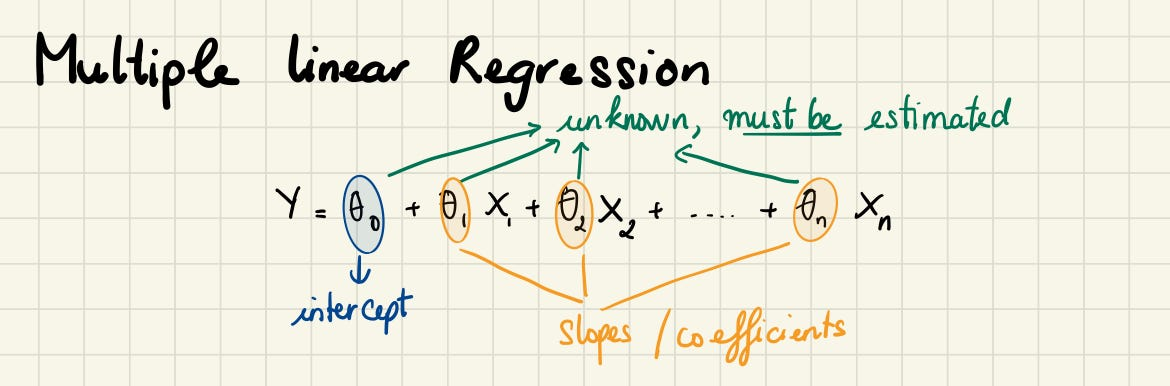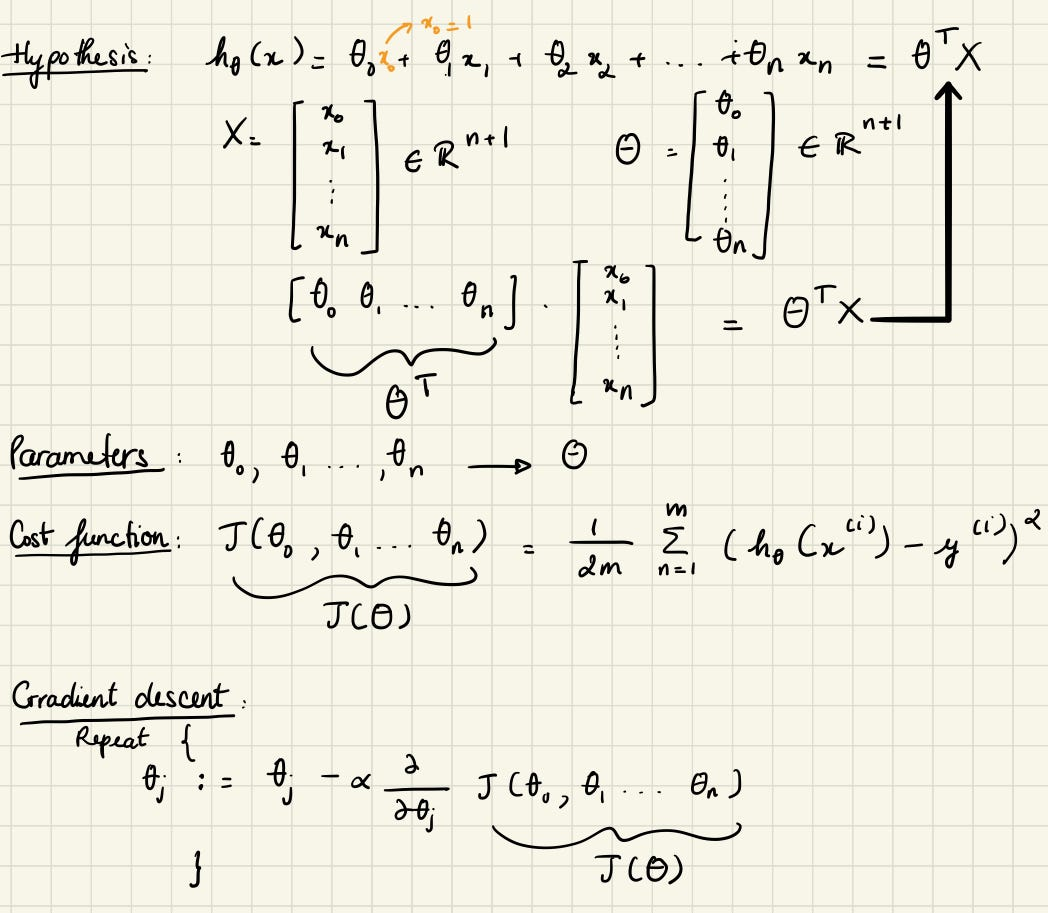Multiple linear regression is what we can use when we have different independent variables. Multiple linear regression looks at the relationships within many information. Instead of just looking at how one baby contributes to the noise in the house (simple linear regression). We can look at the strength of the effect of the independent variables on the dependent variable (which baby is louder, who is more silent, etc…) We can also look at the relationship between babies and the thing we want to predict — how much noise we could have. Fun !!!
当我们具有不同的自变量时，可以使用多元线性回归 。 多元线性回归着眼于许多信息中的关系。 而不是仅仅看一个婴儿如何影响屋子里的噪音(简单的线性回归)。 我们可以查看独立变量对因变量的影响强度(哪个婴儿声音更大，谁更沉默，等等……)我们还可以查看婴儿与我们想要预测的事物之间的关系-多少我们可能会有噪音。 好玩!!!

If two or more explanatory variables have a linear relationship with the dependent variable, the regression is called a multiple linear regression. The multiple linear regression explains the relationship between one continuous dependent variable (y) and two or more independent variables (x1, x2, x3… etc).
如果两个或多个解释变量与因变量具有线性关系，则该回归称为多元线性回归 。 多元线性回归解释了   一个连续因变量( y )和两个或多个自变量   ( x 1， x 2， x 3…等) 。

Multiple linear regression is simple linear regression, but with more relationships
多元线性回归是简单的线性回归，但关系更多

Note: The difference between the simple and multiple linear regression is the number of independent variables. In both cases, there is only a single dependent variable.
注意 ：简单线性回归和多元线性回归之间的差异是自变量的数量。 在这两种情况下，都只有一个因变量。

案例研究： (A case study:)
We have learned all we need to implement multiple linear regression. Now it’s time to see how it works on a dataset. I have learned so much by performing a multiple linear regression in Python. I hope you will learn a thing or two after reading my note.
我们已经了解了实现多元线性回归所需的一切。 现在是时候看看它如何在数据集上工作了。 通过在Python中执行多元线性回归，我学到了很多东西。 希望您阅读我的笔记后能学到一两个东西。
I downloaded the World Happiness Report from Kaggle. You can search on Kaggle for competitions, datasets, and other solutions. In this example, we want to predict the happiness score based on multiple variables.
我从Kaggle下载了《 世界幸福报告》 。 您可以在Kaggle上搜索比赛，数据集和其他解决方案。 在此示例中，我们希望基于多个变量预测幸福分数。
特征分析 (Features Analyzed)
GDP: GDP per capita is a measure of a country’s economic output that accounts for its number of people. GDP ：人均GDP是一国经济产出的量度，该数字是其人口数量的一部分。 Support: Social support means having friends and other people, including family, turning to in times of need or crisis to give you a broader focus and positive self-image. Social support enhances the quality of life and provides a buffer against adverse life events. 支持 ：社会支持意味着有朋友和其他人(包括家人)在需要或危机时求助于您，以给予您更广泛的关注和积极的自我形象。 社会支持提高了生活质量，并为不良生活事件提供了缓冲。 Health: Healthy Life Expectancy is the average number of years that a newborn can expect to live in “full health” — in other words, not hampered by disabling illnesses or injuries. 健康 ：健康的预期寿命是指新生儿可以“完全健康”生活的平均年限，换句话说，不受疾病或伤害致残的影响。 Freedom: Freedom of choice describes an individual’s opportunity and autonomy to perform an action selected from at least two available options, unconstrained by external parties. 自由：选择自由描述了个人执行机会的自主权和自主权，该机会是从至少两个不受外部团体约束的可用选项中选择的。 Generosity: The quality of being kind and generous. 慷慨 ：友善和慷慨的品质。 Corruption: The Corruption Perceptions Index (CPI) is an index published annually by Transparency International since 1995, which ranks countries “by their perceived levels of public sector corruption, as determined by expert assessments and opinion surveys. 腐败 ：腐败感知指数(CPI)是透明国际自1995年以来每年发布的指数，该指数将国家“根据专家评估和意见调查所确定的公共部门腐败程度进行排名”。
导入模块，读取数据集并定义评估表 (Import Modules, Read the Dataset and Define an Evaluation Table)
To do some analysis, we need to set up our environment. First, we import some modules and read the data. The below output is the head of the data, but if you want to see more details, you might try removing # signs in front of the df_15.describe()and df_15.info()
要进行一些分析，我们需要设置环境。 首先，我们导入一些模块并读取数据。 下面的输出是数据的开头，但是如果您想查看更多详细信息，则可以尝试删除df_15.describe()和df_15.info()前面的＃号。
# FOR NUMERICAL ANALYTICSimport numpy as np# TO STORE AND PROCESS DATA IN DATAFRAMEimport pandas as pd# BASIC VISUALIZATION PACKAGEimport matplotlib.pyplot as plt# ADVANCED PLOTINGimport seaborn as seabornInstance# TRAIN TEST SPLITfrom sklearn.model_selection import train_test_split# STATSfrom sklearn import datasets, metricsfrom sklearn.metrics import mean_squared_errorfrom sklearn.linear_model import LinearRegressionfrom sklearn.model_selection import cross_val_score#2015 datadf_15 = pd.read_csv('2015.csv')#df_15.describe()#df_15.info()usecols = ['Rank','Country','Score','GDP','Support',                'Health','Freedom','Generosity','Corruption']df_15.drop(['Region','Standard Error', 'Dystopia Residual'],axis=1,inplace=True) df_15.columns = ['Country','Rank','Score','Support',                'GDP','Health',                'Freedom','Generosity','Corruption']df_15['Year'] = 2015 #add year columndf_15.head()
output.

I only present the code for 2015 data as an example; you could do similar for other years.
我仅以2015年数据的代码为例； 其他年份也可以做类似的事情。
Parts starting with Happiness, Whisker and the Dystopia.Residual are targets, just different targets. Dystopia Residual compares each countries scores to the theoretical unhappiest country in the world. Since the data from the years have a bit of a different naming convention, so we will abstract these to a familiar name.
从Happiness ， Whisker和Dystopia.Residual开始的零件。 Dystopia.Residual是目标，只是不同的目标。 反乌托邦残差将每个国家的得分与世界上理论上最不幸的国家进行比较。 由于这些年份的数据有一些不同的命名约定，因此我们将其抽象为一个熟悉的名称。
target = ['Top','Top-Mid', 'Low-Mid', 'Low' ]target_n = [4, 3, 2, 1]df_15["target"] = pd.qcut(df_15['Rank'], len(target), labels=target)df_15["target_n"] = pd.qcut(df_15['Rank'], len(target), labels=target_n)
Combine all data file to final df
将所有数据文件合并到最终df
# APPENDING ALL TOGUETHERfinaldf = df_15.append([df_16,df_17,df_18,df_19])# finaldf.dropna(inplace = True)#CHECKING FOR MISSING DATAfinaldf.isnull().any()# FILLING MISSING VALUES OF CORRUPTION PERCEPTION WITH ITS MEANfinaldf.Corruption.fillna((finaldf.Corruption.mean()), inplace = True)finaldf.head(10)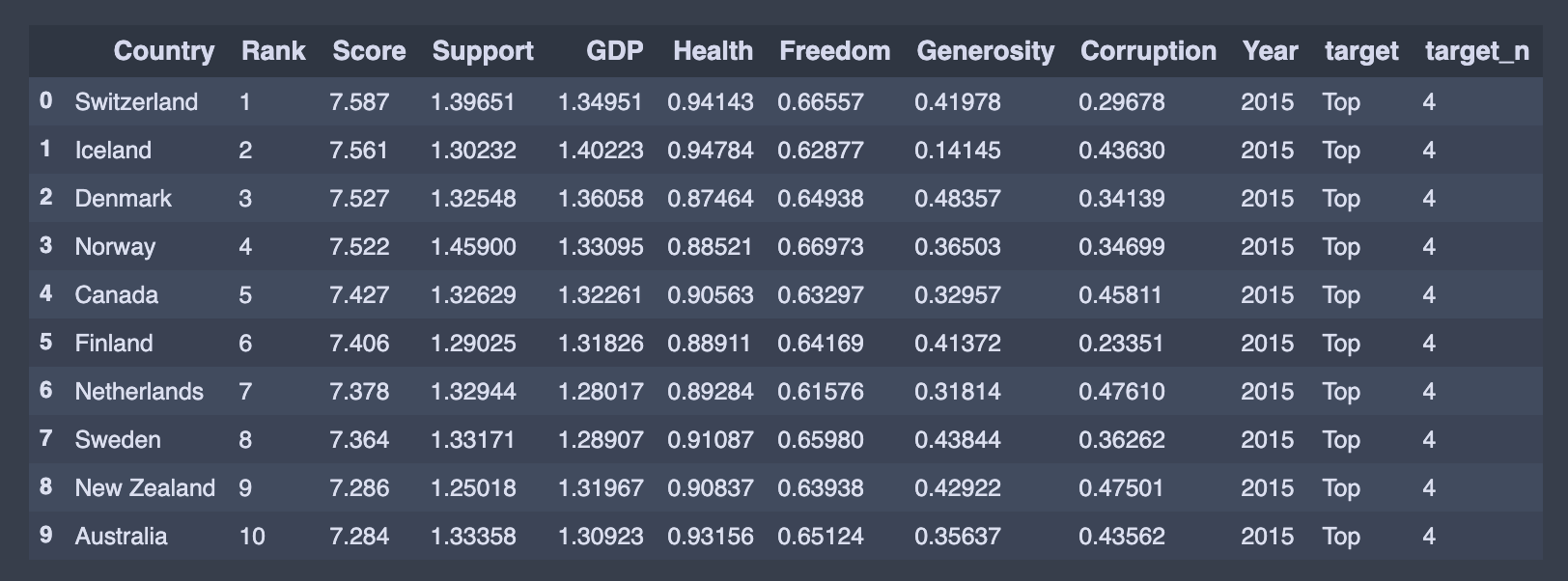We can see the statistical detail of our dataset by using describe() function:
我们可以使用describe()函数查看数据集的统计细节：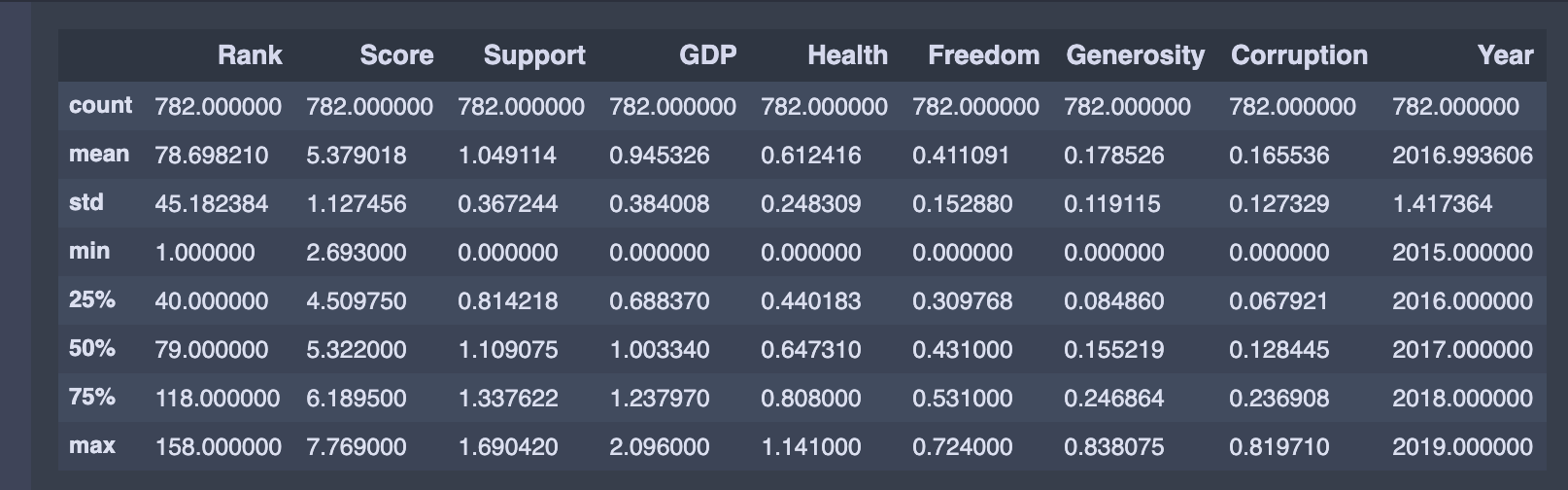Further, we define an empty dataframe. This dataframeincludes Root Mean Squared Error (RMSE), R-squared, Adjusted R-squared, and mean of the R-squared values obtained by the k-Fold Cross-Validation, which are the essential metrics to compare different models. Having an R-squared value closer to one and smaller RMSE means a better fit. In the following sections, we will fill this dataframe with the results.
此外，我们定义了一个空的dataframe 。 此dataframe包括Root Mean Squared Error (RMSE) ， R-squared ， Adjusted R-squared和mean of the R-squared values obtained by the k-Fold Cross-Validation ，这是比较不同模型的基本指标。 R平方值更接近一个较小的RMSE意味着更合适。 在以下各节中，我们将用结果填充此dataframe 。
evaluation = pd.DataFrame({'Model':[],                          'Details':[],                          'Root Mean Squared Error (RMSE)': [],                          'R-squared (training)': [],                          'Adjusted R-squared (training)': [],                          'R-squared (test)':[],                          'Adjusted R-squared(test)':[],                           '5-Fold Cross Validation':[]                        })
定义一个函数来计算调整后的R² (Define a Function to Calculate the Adjusted R²)
R-squared increases when the number of features increases. Because of this, sometimes, a more robust evaluator is preferred to compare the performance between different models. This evaluator is called adjusted R-squared.
当特征数量增加时， R平方增加。 因此，有时最好使用功能更强大的评估器来比较不同模型之间的性能。 该评估器称为调整后的R平方。
The adjusted R-squared compensates for the addition of variables and only increases if the new predictor enhances the model above what would be obtained by probability. Conversely, it will decrease when a predictor improves the model less than what is predicted by chance. The definition of the adjusted R² is:
调整后的R平方会补偿变量的增加，并且只有在新的预测变量将模型增强到高于通过概率获得的模型时，才会增加。 相反，当预测变量对模型的改进少于偶然预测时，它将减少。 调整后的R²的定义为：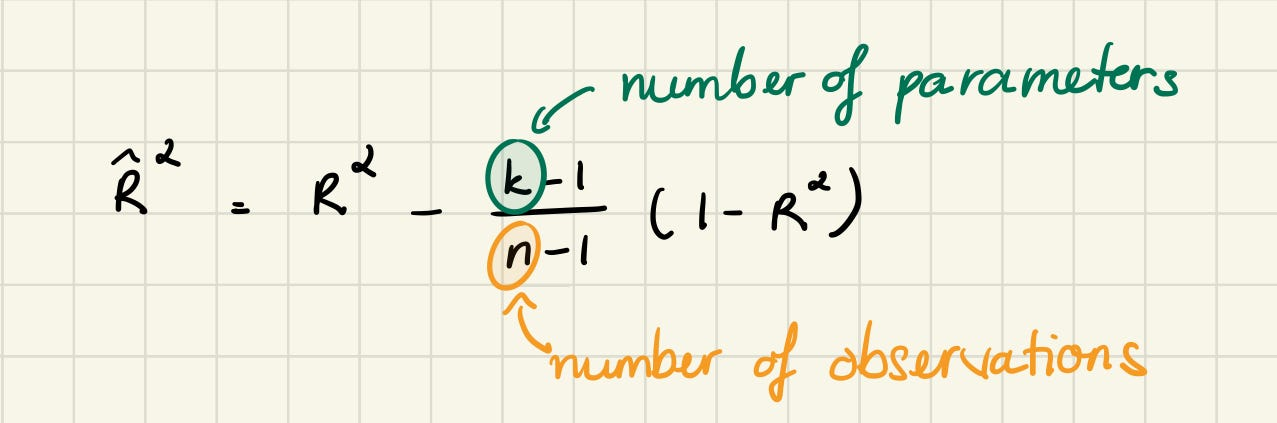def adjustedR2(r2,n,k):    return r2-(k-1)/(n-k)*(1-r2)
创建一个简单的线性回归模型 (Create a Simple Linear Regression Model)
We want to predict happiness score, so our dependent variable here is score. I decided to use GPD as our independent variable, but if you're going to examine the relationship between the happiness score and another feature, you may prefer that feature.
我们想预测幸福分数，因此我们的因变量是score 。 我决定将GPD用作我们的自变量，但是如果您要检查幸福感评分与其他功能之间的关系，则可能更喜欢该功能。
train_data, test_data = train_test_split(finaldf, train_size = 0.8, random_state = 3)lr = LinearRegression()X_train = np.array(train_data['GDP'],                   dtype = pd.Series).reshape(-1,1)y_train = np.array(train_data['Score'], dtype = pd.Series)lr.fit(X_train, y_train)X_test = np.array(test_data['GDP'],                     dtype = pd.Series).reshape(-1,1)y_test = np.array(test_data['Score'], dtype = pd.Series)pred = lr.predict(X_test)#ROOT MEAN SQUARED ERRORrmsesm = float(format(np.sqrt(metrics.mean_squared_error(y_test,pred)),'.3f'))#R-SQUARED (TRAINING)rtrsm = float(format(lr.score(X_train, y_train),'.3f'))#R-SQUARED (TEST)rtesm = float(format(lr.score(X_test, y_test),'.3f'))cv = float(format(cross_val_score(lr,finaldf[['GDP']],finaldf['Score'],cv=5).mean(),'.3f'))print ("Average Score for Test Data: {:.3f}".format(y_test.mean()))print('Intercept: {}'.format(lr.intercept_))print('Coefficient: {}'.format(lr.coef_))print('Happiness score = ',np.round(lr.intercept_,4),      '+',np.round(lr.coef_,4),'* GDP')r = evaluation.shapeevaluation.loc[r] = ['Simple Linear Regression','-',rmsesm,rtrsm,'-',rtesm,'-',cv]evaluation
By using these values and the below definition, we can estimate the happiness score manually. The equation we use for our estimations is called hypothesis function and defined as:
通过使用这些值和以下定义，我们可以手动估算幸福感评分。 我们用于估计的方程称为假设函数，定义为：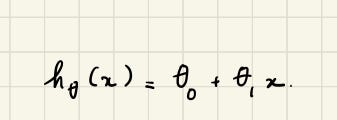We also printed the intercept and coefficient for the simple linear regression.
我们还为简单的线性回归打印了截距和系数。
Result

Since we have just two dimensions at the simple regression, it is easy to draw it. The below chart determines the result of the simple regression. It does not look like a perfect fit, but when we work with real-world datasets, having an ideal fit is not easy.
由于在简单回归中只有二维，因此绘制起来很容易。 下图确定了简单回归的结果。 它看起来并不完美，但是当我们处理真实数据集时，要实现理想的拟合并不容易。
seabornInstance.set_style(style='whitegrid')plt.figure(figsize=(12,6))plt.scatter(X_test,y_test,color='blue',label="Data", s = 12)plt.plot(X_test,lr.predict(X_test),color="red",label="Predicted Regression Line")plt.xlabel("GDP per Captita", fontsize=15)plt.ylabel("Happiness Score", fontsize=15)plt.xticks(fontsize=13)plt.yticks(fontsize=13)plt.legend()plt.gca().spines['right'].set_visible(False)plt.gca().spines['top'].set_visible(False)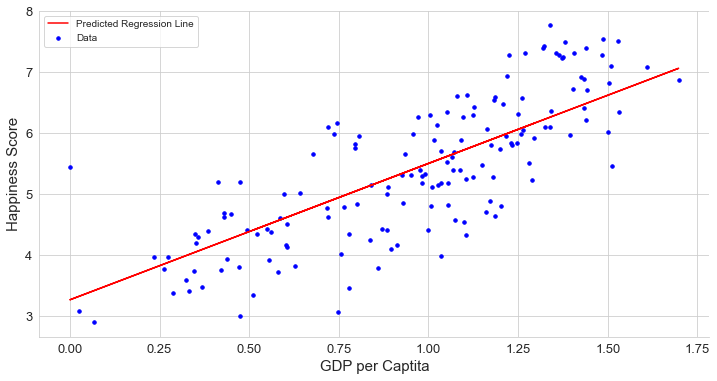创建多元线性回归模型 (Create a Multiple Linear Regression Model)
We used a simple linear regression and found a poor fit. To improve this model, we want to add more features. Now, it is time to create some complex models.
我们使用了简单的线性回归，发现拟合度很差。 为了改进此模型，我们想添加更多功能。 现在，该创建一些复杂的模型了。
We determined features at first by looking at the previous sections and used them in our first multiple linear regression. As in the simple regression, we printed the coefficients which the model uses for the predictions. However, this time we must use the below definition for multiple linear regression:
我们首先通过查看前面的部分来确定特征，并将其用于我们的第一个多元线性回归中。 与简单回归一样，我们打印了模型用于预测的系数。 但是，这次我们必须对多元线性回归使用以下定义：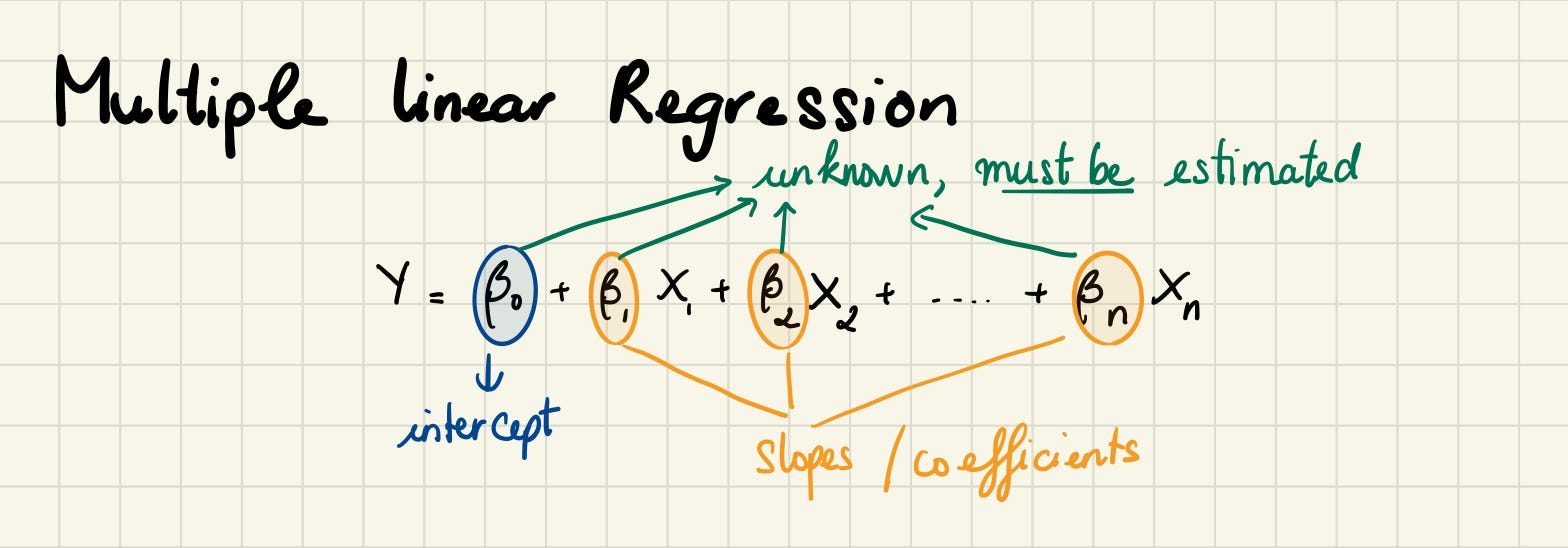We create a model with all features.
我们创建具有所有功能的模型。
# MULTIPLE LINEAR REGRESSION 1train_data_dm,test_data_dm = train_test_split(finaldf,train_size = 0.8,random_state=3)independent_var = ['GDP','Health','Freedom','Support','Generosity','Corruption']complex_model_1 = LinearRegression()complex_model_1.fit(train_data_dm[independent_var],train_data_dm['Score'])print('Intercept: {}'.format(complex_model_1.intercept_))print('Coefficients: {}'.format(complex_model_1.coef_))print('Happiness score = ',np.round(complex_model_1.intercept_,4),      '+',np.round(complex_model_1.coef_,4),'∗ Support',      '+',np.round(complex_model_1.coef_,4),'* GDP',       '+',np.round(complex_model_1.coef_,4),'* Health',      '+',np.round(complex_model_1.coef_,4),'* Freedom',       '+',np.round(complex_model_1.coef_,4),'* Generosity',      '+',np.round(complex_model_1.coef_,4),'* Corrption')pred = complex_model_1.predict(test_data_dm[independent_var])rmsecm = float(format(np.sqrt(metrics.mean_squared_error(                       test_data_dm['Score'],pred)),'.3f'))rtrcm = float(format(complex_model_1.score(                        train_data_dm[independent_var],                        train_data_dm['Score']),'.3f'))artrcm = float(format(adjustedR2(complex_model_1.score(                            train_data_dm[independent_var],                            train_data_dm['Score']),                            train_data_dm.shape,                            len(independent_var)),'.3f'))rtecm = float(format(complex_model_1.score(                        test_data_dm[independent_var],                        test_data_dm['Score']),'.3f'))artecm = float(format(adjustedR2(complex_model_1.score(                        test_data_dm[independent_var],test_data['Score']),                        test_data_dm.shape,                        len(independent_var)),'.3f'))cv = float(format(cross_val_score(complex_model_1,                    finaldf[independent_var],                    finaldf['Score'],cv=5).mean(),'.3f'))r = evaluation.shapeevaluation.loc[r] = ['Multiple Linear Regression-1','selected features',rmsecm,rtrcm,artrcm,rtecm,artecm,cv]evaluation.sort_values(by = '5-Fold Cross Validation', ascending=False)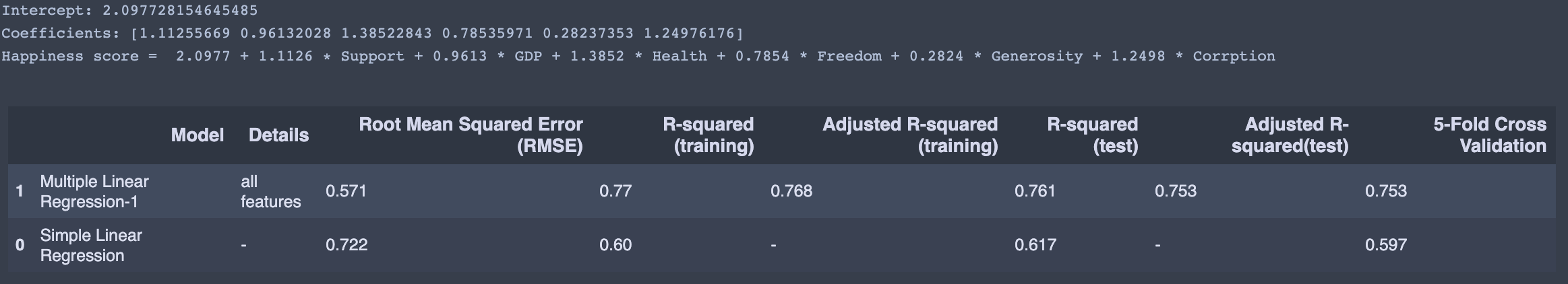The population regression line for n independent variables x(n) is defined to beHappiness score = 2.0977 + 1.1126 ∗ Support + 0.9613 * GDP + 1.3852 * Health + 0.7854 * Freedom + 0.2824 * Generosity + 1.2498 * Corrption . This line describes how thehappiness score changes with the independent variables (Support, GDP, Health, Freedom, Generosity, and Corruption)
n个自变量x(n)的总体回归线定义为Happiness score = 2.0977 + 1.1126 ∗ Support + 0.9613 * GDP + 1.3852 * Health + 0.7854 * Freedom + 0.2824 * Generosity + 1.2498 * Corrption 。 该行描述happiness score如何随独立变量( Support ， GDP ， Health ， Freedom ， Generosity和Corruption )变化。
Check Out the Correlation Among Independent Variables
检查自变量之间的相关性
mask = np.zeros_like(finaldf[usecols].corr(), dtype=np.bool) mask[np.triu_indices_from(mask)] = Truef, ax = plt.subplots(figsize=(16, 12))plt.title('Pearson Correlation Matrix',fontsize=25)seabornInstance.heatmap(finaldf[usecols].corr(),                        linewidths=0.25,vmax=0.7,square=True,cmap="Blues",             linecolor='w',annot=True,annot_kws={"size":8},mask=mask,cbar_kws={"shrink": .9});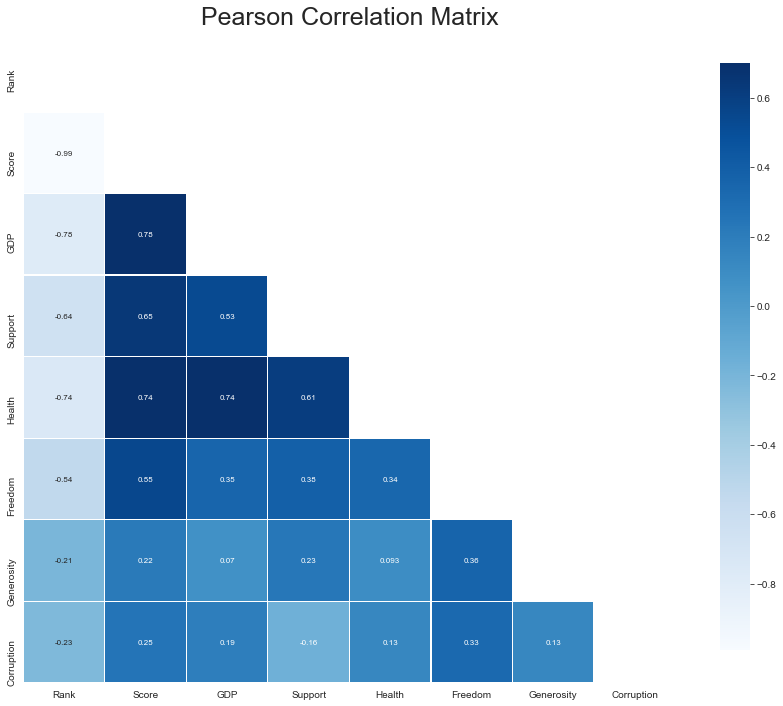It looks like GDP, Health, and Support are strongly correlated with the Happiness score. Freedom and correlates quite well with the, however, Freedom connects quite well with all data. Corruption still has a mediocre correlation with the Happiness score.
看起来GDP ， Health和Support与Happiness score密切相关。 Freedom与Freedom关联性很好，但是Freedom与所有数据的关联性很好。 Corruption与Happiness score.之间仍存在中等程度的相关性Happiness score.
使用梯度下降的多元线性回归 (Multiple Linear Regression using Gradient Descent)
We can implement various linear regression using gradient descent.
我们可以使用梯度下降来实现各种线性回归。
X = train_data_dm[independent_var]y = train_data_dm['Score']''' This function takes the features as input and returns the normalized values, the mean, as well  as the standard deviation for each feature.  '''def featureNormalize(X):    mu = np.mean(X) # Define the mean    sigma = np.std(X) # Define the standard deviation.    X_norm = (X - mu)/sigma # Scaling function.    return X_norm, mu, sigmam = len(y) ## length of the training dataX = np.hstack((np.ones([m,1]), X)) # Append the bias term (field containing all ones) to X.y = np.array(y).reshape(-1,1) # reshape y to mx1 arraytheta = np.zeros([7,1]) # Initialize theta (the coefficient) to a 3x1 zero vector.'''  This function takes in the the values for  the training set as well as initial values  of theta and returns the cost(J). '''def cost_function(X,y, theta):    h = X.dot(theta) # The hypothesis    J = 1/(2*m)*(np.sum((h-y)**2)) # Implementing the cost function    return J'''    This function takes in the values of the set,     as well the intial theta values(coefficients), the     learning rate, and the number of iterations. The output     will be the a new set of coefficeients(theta), optimized     for making predictions, as well as the array of the cost     as it depreciates on each iteration.'''num_iters = 2000 # Initialize the iteration parameter.alpha = 0.01 # Initialize the learning rate.def gradientDescentMulti(X, y, theta, alpha, iterations):    m = len(y)    J_history = []    for _ in range(iterations):        temp = np.dot(X, theta) - y        temp = np.dot(X.T, temp)        theta = theta - (alpha/m) * temp        J_history.append(cost_function(X, y, theta)) # Append the        cost to the J_history array    return theta, J_historytheta , J_history = gradientDescentMulti(X, y, theta, alpha, num_iters)print('Happiness score = ',np.round(theta,4),        '+',np.round(theta,4),'∗ Support',      '+',np.round(theta,4),'* GDP',       '+',np.round(theta,4),'* Health',      '+',np.round(theta,4),'* Freedom',       '+',np.round(theta,4),'* Generosity',      '+',np.round(theta,4),'* Corrption')

result

The code in this note is available on Github.
该注释中的代码可在Github上获得 。
我们做到了！ (We did it!)
In this note, we learned the basics of multiple linear regression and its implementation in Python. We will discuss logistic regression next.
在本文中，我们学习了多元线性回归的基础知识及其在Python中的实现。 接下来我们将讨论逻辑回归。
Keep on learning!
继续学习！
meme
米姆

翻译自: https://medium.com/nothingaholic/multiple-linear-regression-with-python-22e2087cd628

多元线性回归 python

展开全文python 机器学习 人工智能
• 线性回归模型属于经典的统计学模型，该模型的应用场景是根据已知的变量（自变量）来预测某个连续的数值变量（因变量）。例如，餐厅根据每天的营业数据（包括菜谱价格、就餐人数、预定人数、特价菜折扣等）预测就餐...
• 机器学习之多元线性回归1.多元线性回归 在上一篇简单线性回归中我们已经介绍简单和多元线性回归的概念划分 1.多元线性回归机器学习 python
• 多元线性回归建模 文章大纲 (Article Outline) Dataset description 数据集描述 Exploratory data analysis 探索性数据分析 A simple linear regression model fitting 简单的线性回归模型拟合 Model interpretation...机器学习 人工智能 python 深度学习
• 1、线性回归概述 2、数据准备 3、数据探索 3.1简单的数据清洗 3.2 图形分析数据 3.2.1 盒形图的离群点分析，需要根据四分位距去掉部分数据点。 3.2.2 密度图进行分布分析 3.2.3 散点图相关分析 4、数据...机器学习 数据分析 julia
• 当存在分类变量时，需要首先处理成多个哑变量，例如将年龄分成三个:age<18, age18_60, age>60。 然后在SPSS里纳入除了ref的所有哑变量，例如我们要将18-60作为参考组，就纳入age<18和age>60，不要纳入...
•损失函数 梯度下降
• 什么是回归分析？ 相关性 ≠ 因果性 ...[外链图片转存失败,源站可能有防盗链机制,建议将图片保存下来直接上传(img-NKvCz36V-1600444325643)(figures/多元线性回归模型/image-20200824222649255.数学建模
• 多元回归的求解比简单线性回归复杂但是思路是相同的，运用最小二乘法进行相应的求解，这里不再进行展开。 python实现的小例子： 问题：如故一个运输任务是跑102英里，运输了6次，预计多少小时？ from numpy ...
• 线性回归是利用数理统计中回归分析，来确定两种或两种以上变量间相互依赖的定量关系的一种统计分析方法，运用十分广泛。其表达形式为y = w’x+e，e为误差服从均值为0的正态分布。 一元与多元 回归分析中，只包括一...
• 线性回归模型属于经典的统计学模型，该模型的应用场景是根据已知的变量（自变量）来预测某个连续的数值变量（因变量）。例如，餐厅根据每天的营业数据（包括菜谱价格、就餐人数、预定人数、特价菜折扣等）预测就餐...
• 多简单线性回归相比，多元线性回归不过是多了几个自变量x机器学习 回归问题
• 多元线性回归的基本表达式在多元线性回归中会有多个解释变量：预测解释变量的估计方程如下：注：额外的假设条件①解释变量之间不能存在太强的线性相关关系(一般ρ<0.7)②其他条件与一元线性回归类似。2.回归方程...
• 多元线性回归分析 回归分析是数据分析中最基础也是最重要的分析工具，绝大多数的数据分析问题，都可以使用回归的思想来解决。回归分析的任务就是，通过研究自变量X和因变量Y的相关关系，尝试去解释Y的形成机制，进而...
• 回归一直是个很重要的主题。因为在数据分析的领域里边，模型重要的也是主要...本文主要讨论多元线性回归（包括一般多元回归，含有虚拟变量的多元回归，以及一点广义差分的知识）。请大家不要觉得本人偷奸耍滑，居然只有回归
• 　在统计学中，线性回归是利用称为线性回归方程的最小二乘函数对一个或多个自变量和因变量之间关系进行建模的一种回归分析。这种函数是一个或多个称为回归系数的模型参数的线性组合。一个带有一个自变量线性回归...机器学习
• 多元线性回归：含有多个特征及多个自变量（输入） 公式： 参数： 损失函数： 梯度下降： Repeat{ (j=0,1,2,3,4,...,n) } 下面是未使用sklearn的代码 import numpy as np import matplotlib.pyplot as ...
• 假定被解释变量与多个解释变量之间具有线性关系，是解释变量的多元线性函数，称为多元线性回归模型。即  (1.1) 其中为被解释变量，为个解释变量，为个未知参数，为随机误差项。 被解释变量的期望值与解释变量的...机器学习
• 多元线性回归模型评估You’ve got a sample dataset and just finished working on a machine learning algorithm using the linear regression model. But now, you are wondering whether or not your analysis ...机器学习 python 人工智能 深度学习
• 线性回归1.1 基本形式1.2 最小二乘法推导2. Sklearn 实现参考资料 相关文章：机器学习 | 回归评估指标 1. 线性回归 线性回归，又称普通最小二乘法（Ordinary Least Squares, OLS），是回归问题最简单也最经典的...机器学习 监督学习 Python Sklearn
• 线性回归，前面用Python从底层一步一个脚印用两种方法实现了回归拟合。在这个高级语言层出不穷的年代，这样做显然不明智，所以我考虑用优秀的数据分析工具——R语言（不敢说最...python 机器学习
• 定义：回归分析中，含有两个或者两个以上自变量，称为多元回归，若自变量系数为1，则此回归为多元线性回归。 （特殊的：自变量个数为1个，为一元线性回归）多元线性回归模型如下所示： 如上图所示，一元线性...机器学习
• 多元线性回归本质上与单元变量线性回归没太大区别” 增添更多特征后，我们引入一系列新的注释： 引入多元变量后，我们直接把原来的函数 简写为 多元线性回归 梯度下降 多元变量的线性回归的梯度下降与一元的没...机器学习
• 前言：当影响因变量的因素是多个时候，这种一个变量同时与多个变量的回归问题就是多元回归，分为：多元线性回归和多元非线性回归。线性回归(Linear regressions)和逻辑回归(Logistic regressions)是人们学习算法的第...机器学习算法
• 多元线性回归 一个多元线性回归试图通过对观测数据拟合线性方程来模拟两个或多个特征与响应之间的关系。执行多重线性回归的步骤几乎与简单线性回归的步骤相似。 差异在于评价。你可以用它来找出哪个因素对预测输出...机器学习 python...